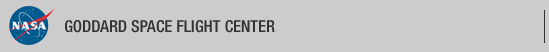+ Visit NASA.gov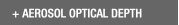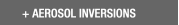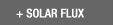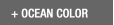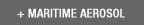Web Site Feature AERONET Data Synergy Tool - Access Earth Science data sets for AERONET sites+Home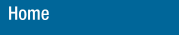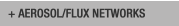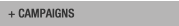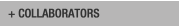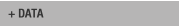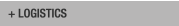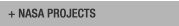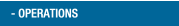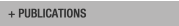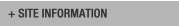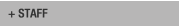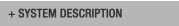AERONET DATA ACCESS

AERONET Site Lists (V3)

+ All Lists# Report of DCP transmissions

 Status Legend

AERONET No 6 [1317E33A] - 27 msg rcvd (4233 b) site : GSFC (Last Tx is before schedule by 0 min 3 sec
27 messages stepped outside their assigned time window)
AERONET No 26 [1316E1C0] - 27 msg rcvd (4616 b) site : GSFC (Last Tx is late by 0 min 1 sec)
AERONET No 28 [13101F58] - 27 msg rcvd (17954 b) site : White_Sands_HELSTF (Last Tx is precisely on time)
AERONET No 59 [131A03AE] - 26 msg rcvd (3727 b) site : Sevilleta (Last Tx is precisely on time)
AERONET No 134  - 27 msg rcvd (1917 b) site : GSFC (Last Tx is before schedule by 0 min 3 sec
27 messages stepped outside their assigned time window)
AERONET No 163  - 27 msg rcvd (16204 b) site : UCSB (Last Tx is precisely on time)
AERONET No 196 [1319A458] - 27 msg rcvd (18394 b) site : Rio_Branco (Last Tx is precisely on time)
AERONET No 306 [131A40A4] - 27 msg rcvd (5266 b) site : Easton-MDE (Last Tx is precisely on time , 33b)
AERONET No 307 [1318152C] - 26 msg rcvd (9120 b) site : Ames (Last Tx is late by 0 min 1 sec , 6b)
AERONET No 317 [131982B4] - 27 msg rcvd (17670 b) site : Tudor_Hill (Last Tx is precisely on time)
AERONET No 319 [131820B6] - 26 msg rcvd (6787 b) site : Trelew (Last Tx is late by 0 min 1 sec 230s)
AERONET No 330 [131481D2] - 27 msg rcvd (5241 b) site : GSFC (Last Tx is late by 0 min 1 sec)
AERONET No 365 [131A53D2] - 26 msg rcvd (14068 b) site : Ji_Parana_SE (Last Tx is precisely on time , 22b)
AERONET No 374 [131713BE] - 27 msg rcvd (21230 b) site : TABLE_MOUNTAIN_CA (Last Tx is precisely on time)
AERONET No 409 [131AA356] - 23 msg rcvd (4655 b) site : Mingo (Last Tx is late by 0 min 1 sec)
AERONET No 601 [1319E752] - 24 msg rcvd (2688 b) site : CCNY ()
AERONET No 624 [131AE05C] - 27 msg rcvd (3090 b) site : CUIABA-MIRANDA ()
AERONET No 635 [1319D2C8] - 27 msg rcvd (10721 b) site : Alta_Floresta (Last Tx is precisely on time)
AERONET No 813 [1310118A] - 26 msg rcvd (20556 b) site : Yuma (Last Tx is precisely on time , 3b)

# Aeronet No 6 [1317E33A] (GSFC)

Last Tx is before schedule by 0 min 3 sec
27 messages stepped outside their assigned time window

10:12:2019,10:41:47 (UTC) 'G' (DCP total: 87) Cimel : 6 time corr. = -26 sec (DCP Volt = 13.00 Lat= 38.993 Long= -76.840(0.1 km from GSFC))
10:12:2019,11:41:47 (UTC) 'G' (DCP total: 87) Cimel : 6 time corr. = -25 sec (DCP Volt = 13.00 Lat= 38.993 Long= -76.840(0.1 km from GSFC))
10:12:2019,12:41:47 (UTC) 'G' (DCP total: 87) Cimel : 6 time corr. = -25 sec (DCP Volt = 13.00 Lat= 38.993 Long= -76.841(0.1 km from GSFC))
10:12:2019,13:41:47 (UTC) 'G' (DCP total: 313) Cimel : 6 time corr. = -26 sec (DCP Volt = 13.00 Lat= 38.993 Long= -76.840(0.0 km from GSFC)) staD nsu staD
10:12:2019,14:41:47 (UTC) 'G' (DCP total: 468) Cimel : 6 time corr. = -25 sec (DCP Volt = 13.00 Lat= 38.992 Long= -76.840(0.1 km from GSFC)) nsu staD nsu staD nsu staD nsu stah
10:12:2019,15:41:47 (UTC) 'G' (DCP total: 339) Cimel : 6 time corr. = -25 sec (DCP Volt = 13.00 Lat= 38.992 Long= -76.840(0.1 km from GSFC)) stah stah stah stah stah stah stah stah stah stah stah stah
10:12:2019,16:41:47 (UTC) 'G' (DCP total: 339) Cimel : 6 time corr. = -26 sec (DCP Volt = 13.00 Lat= 38.992 Long= -76.840(0.1 km from GSFC)) stah stah stah stah stah stah stah stah stah stah stah stah
10:12:2019,17:41:47 (UTC) 'G' (DCP total: 339) Cimel : 6 time corr. = -25 sec (DCP Volt = 13.00 Lat= 38.992 Long= -76.840(0.1 km from GSFC)) stah stah stah stah stah stah stah stah stah stah stah stah
10:12:2019,18:41:47 (UTC) 'G' (DCP total: 339) Cimel : 6 time corr. = -25 sec (DCP Volt = 13.00 Lat= 38.992 Long= -76.840(0.1 km from GSFC)) stah stah stah stah stah stah stah stah stah stah stah stah
10:12:2019,19:41:47 (UTC) 'G' (DCP total: 339) Cimel : 6 time corr. = -26 sec (DCP Volt = 13.00 Lat= 38.992 Long= -76.840(0.1 km from GSFC)) stah stah stah stah stah stah stah stah stah stah stah stah
10:12:2019,20:41:47 (UTC) 'G' (DCP total: 339) Cimel : 6 time corr. = -25 sec (DCP Volt = 13.00 Lat= 38.992 Long= -76.840(0.1 km from GSFC)) stah stah stah stah stah stah stah stah stah stah stah stah
10:12:2019,21:41:47 (UTC) 'G' (DCP total: 108) Cimel : 6 time corr. = -25 sec (DCP Volt = 13.00 Lat= 38.993 Long= -76.840(0.0 km from GSFC)) stah
10:12:2019,22:41:47 (UTC) 'G' (DCP total: 87) Cimel : 6 time corr. = -26 sec (DCP Volt = 13.00 Lat= 38.993 Long= -76.840(0.0 km from GSFC))
10:12:2019,23:41:47 (UTC) 'G' (DCP total: 87) Cimel : 6 time corr. = -25 sec (DCP Volt = 13.00 Lat= 38.992 Long= -76.840(0.1 km from GSFC))
11:12:2019,00:41:47 (UTC) 'G' (DCP total: 87) Cimel : 6 time corr. = -26 sec (DCP Volt = 13.00 Lat= 38.993 Long= -76.840(0.0 km from GSFC))
11:12:2019,01:41:47 (UTC) 'G' (DCP total: 87) Cimel : 6 time corr. = -27 sec (DCP Volt = 13.00 Lat= 38.992 Long= -76.840(0.0 km from GSFC))
11:12:2019,02:41:47 (UTC) 'G' (DCP total: 87) Cimel : 6 time corr. = -27 sec (DCP Volt = 13.00 Lat= 38.992 Long= -76.840(0.0 km from GSFC))
11:12:2019,03:41:47 (UTC) 'G' (DCP total: 87) Cimel : 6 time corr. = -26 sec (DCP Volt = 13.00 Lat= 38.993 Long= -76.840(0.0 km from GSFC))
11:12:2019,04:41:47 (UTC) 'G' (DCP total: 87) Cimel : 6 time corr. = -27 sec (DCP Volt = 13.00 Lat= 38.993 Long= -76.840(0.0 km from GSFC))
11:12:2019,05:41:47 (UTC) 'G' (DCP total: 87) Cimel : 6 time corr. = -27 sec (DCP Volt = 13.00 Lat= 38.992 Long= -76.840(0.0 km from GSFC))
11:12:2019,06:41:47 (UTC) 'G' (DCP total: 87) Cimel : 6 time corr. = -26 sec (DCP Volt = 13.00 Lat= 38.993 Long= -76.840(0.0 km from GSFC))
11:12:2019,07:41:47 (UTC) 'G' (DCP total: 87) Cimel : 6 time corr. = -27 sec (DCP Volt = 13.00 Lat= 38.993 Long= -76.840(0.0 km from GSFC))
11:12:2019,09:41:47 (UTC) 'G' (DCP total: 87) Cimel : 6 time corr. = -26 sec (DCP Volt = 13.00 Lat= 38.993 Long= -76.841(0.1 km from GSFC))
11:12:2019,10:41:47 (UTC) 'G' (DCP total: 87) Cimel : 6 time corr. = -27 sec (DCP Volt = 13.00 Lat= 38.993 Long= -76.841(0.1 km from GSFC))
11:12:2019,11:41:47 (UTC) 'G' (DCP total: 87) Cimel : 6 time corr. = -27 sec (DCP Volt = 13.00 Lat= 38.993 Long= -76.841(0.1 km from GSFC))
11:12:2019,12:41:47 (UTC) 'G' (DCP total: 87) Cimel : 6 time corr. = -26 sec (DCP Volt = 13.00 Lat= 38.992 Long= -76.841(0.1 km from GSFC))
11:12:2019,13:41:47 (UTC) 'G' (DCP total: 263) Cimel : 6 time corr. = -27 sec (DCP Volt = 13.00 Lat= 38.993 Long= -76.840(0.0 km from GSFC)) stah staD nsu stah stah

# Aeronet No 26 [1316E1C0] (GSFC)

Last Tx is late by 0 min 1 sec

10:12:2019,10:32:41 (UTC) 'G' (DCP total: 89) Cimel : 26 time corr. = -1 sec (DCP Volt = 12.80 Lat= 38.993 Long= -76.840(0.0 km from GSFC))
10:12:2019,11:32:41 (UTC) 'G' (DCP total: 89) Cimel : 26 time corr. = -2 sec (DCP Volt = 12.80 Lat= 38.993 Long= -76.840(0.0 km from GSFC))
10:12:2019,12:32:41 (UTC) 'G' (DCP total: 89) Cimel : 26 time corr. = -1 sec (DCP Volt = 12.80 Lat= 38.993 Long= -76.840(0.0 km from GSFC))
10:12:2019,13:32:41 (UTC) 'G' (DCP total: 605) Cimel : 26 time corr. = -1 sec (DCP Volt = 12.80 Lat= 38.993 Long= -76.840(0.0 km from GSFC)) staA ssk staA ssk staA ssk staA
10:12:2019,14:32:41 (UTC) 'G' (DCP total: 691) Cimel : 26 time corr. = -3 sec (DCP Volt = 12.80 Lat= 38.992 Long= -76.840(0.0 km from GSFC)) ssk staA ssk staA ssk staB ssk staF nsu staD nsu
10:12:2019,15:32:41 (UTC) 'G' (DCP total: 173) Cimel : 26 time corr. = -3 sec (DCP Volt = 12.80 Lat= 38.992 Long= -76.840(0.0 km from GSFC)) stah stah stah stah
10:12:2019,16:32:41 (UTC) 'G' (DCP total: 173) Cimel : 26 time corr. = -1 sec (DCP Volt = 12.80 Lat= 38.993 Long= -76.840(0.0 km from GSFC)) stah stah stah stah
10:12:2019,17:32:41 (UTC) 'G' (DCP total: 401) Cimel : 26 time corr. = -3 sec (DCP Volt = 13.00 Lat= 38.993 Long= -76.840(0.0 km from GSFC)) staD nsu stah staF nsu staD nsu
10:12:2019,18:32:41 (UTC) 'G' (DCP total: 401) Cimel : 26 time corr. = -3 sec (DCP Volt = 13.00 Lat= 38.993 Long= -76.840(0.0 km from GSFC)) staD nsu staD nsu staF nsu stah
10:12:2019,19:32:41 (UTC) 'G' (DCP total: 173) Cimel : 26 time corr. = -3 sec (DCP Volt = 13.00 Lat= 38.993 Long= -76.840(0.0 km from GSFC)) stah stah stah stah
10:12:2019,20:32:41 (UTC) 'G' (DCP total: 302) Cimel : 26 time corr. = -3 sec (DCP Volt = 12.90 Lat= 38.993 Long= -76.840(0.0 km from GSFC)) stah stah stah stah staA ssk
10:12:2019,21:32:41 (UTC) 'G' (DCP total: 173) Cimel : 26 time corr. = -3 sec (DCP Volt = 12.80 Lat= 38.993 Long= -76.840(0.0 km from GSFC)) stah stah stah stah
10:12:2019,22:32:41 (UTC) 'G' (DCP total: 89) Cimel : 26 time corr. = -3 sec (DCP Volt = 12.80 Lat= 38.993 Long= -76.840(0.0 km from GSFC))
10:12:2019,23:32:41 (UTC) 'G' (DCP total: 89) Cimel : 26 time corr. = -3 sec (DCP Volt = 12.80 Lat= 38.993 Long= -76.840(0.0 km from GSFC))
11:12:2019,00:32:41 (UTC) 'G' (DCP total: 89) Cimel : 26 time corr. = -3 sec (DCP Volt = 12.80 Lat= 38.993 Long= -76.840(0.0 km from GSFC))
11:12:2019,01:32:41 (UTC) 'G' (DCP total: 89) Cimel : 26 time corr. = -3 sec (DCP Volt = 12.80 Lat= 38.993 Long= -76.840(0.0 km from GSFC))
11:12:2019,02:32:41 (UTC) 'G' (DCP total: 89) Cimel : 26 time corr. = -3 sec (DCP Volt = 12.80 Lat= 38.993 Long= -76.840(0.0 km from GSFC))
11:12:2019,03:32:41 (UTC) 'G' (DCP total: 89) Cimel : 26 time corr. = -3 sec (DCP Volt = 12.80 Lat= 38.993 Long= -76.840(0.0 km from GSFC))
11:12:2019,04:32:41 (UTC) 'G' (DCP total: 89) Cimel : 26 time corr. = -3 sec (DCP Volt = 12.80 Lat= 38.993 Long= -76.840(0.0 km from GSFC))
11:12:2019,05:32:41 (UTC) 'G' (DCP total: 89) Cimel : 26 time corr. = -3 sec (DCP Volt = 12.80 Lat= 38.993 Long= -76.840(0.0 km from GSFC))
11:12:2019,06:32:41 (UTC) 'G' (DCP total: 89) Cimel : 26 time corr. = -3 sec (DCP Volt = 12.80 Lat= 38.993 Long= -76.840(0.0 km from GSFC))
11:12:2019,07:32:41 (UTC) 'G' (DCP total: 89) Cimel : 26 time corr. = -3 sec (DCP Volt = 12.80 Lat= 38.993 Long= -76.840(0.0 km from GSFC))
11:12:2019,09:32:41 (UTC) 'G' (DCP total: 89) Cimel : 26 time corr. = -3 sec (DCP Volt = 12.70 Lat= 38.992 Long= -76.840(0.0 km from GSFC))
11:12:2019,10:32:41 (UTC) 'G' (DCP total: 89) Cimel : 26 time corr. = -3 sec (DCP Volt = 12.70 Lat= 38.993 Long= -76.840(0.0 km from GSFC))
11:12:2019,11:32:41 (UTC) 'G' (DCP total: 89) Cimel : 26 time corr. = -3 sec (DCP Volt = 12.70 Lat= 38.993 Long= -76.840(0.0 km from GSFC))
11:12:2019,12:32:41 (UTC) 'G' (DCP total: 89) Cimel : 26 time corr. = -3 sec (DCP Volt = 12.70 Lat= 38.992 Long= -76.840(0.0 km from GSFC))
11:12:2019,13:32:41 (UTC) 'G' (DCP total: 497) Cimel : 26 time corr. = -3 sec (DCP Volt = 12.70 Lat= 38.993 Long= -76.840(0.0 km from GSFC)) stah staA ssk staA ssk staA ssk

# Aeronet No 28 [13101F58] (White_Sands_HELSTF)

Last Tx is precisely on time

10:12:2019,10:12:21 (UTC) 'G' (DCP total: 88) Cimel : 28 time corr. = 1 sec (DCP Volt = 12.20 Lat= 32.635 Long= -106.339(0.1 km from White_Sands_HELSTF))
10:12:2019,11:12:21 (UTC) 'G' (DCP total: 88) Cimel : 28 time corr. = 0 sec (DCP Volt = 12.20 Lat= 32.635 Long= -106.339(0.1 km from White_Sands_HELSTF))
10:12:2019,12:12:21 (UTC) 'G' (DCP total: 88) Cimel : 28 time corr. = 0 sec (DCP Volt = 12.20 Lat= 32.635 Long= -106.339(0.1 km from White_Sands_HELSTF))
10:12:2019,13:12:21 (UTC) 'G' (DCP total: 88) Cimel : 28 time corr. = 1 sec (DCP Volt = 12.20 Lat= 32.635 Long= -106.339(0.1 km from White_Sands_HELSTF))
10:12:2019,14:12:21 (UTC) 'G' (DCP total: 88) Cimel : 28 time corr. = 0 sec (DCP Volt = 12.20 Lat= 32.635 Long= -106.339(0.1 km from White_Sands_HELSTF))
10:12:2019,15:12:21 (UTC) 'G' (DCP total: 573) Cimel : 28 time corr. = 0 sec (DCP Volt = 12.20 Lat= 32.635 Long= -106.339(0.1 km from White_Sands_HELSTF)) staA nsu staA nsu staA nsu staA nsu staA
10:12:2019,16:12:21 (UTC) 'G' (DCP total: 1212) Cimel : 28 time corr. = 1 sec (DCP Volt = 12.40 Lat= 32.635 Long= -106.339(0.1 km from White_Sands_HELSTF)) nsu staA nsu staB nsu staA nsu staA nsu staA nsu staA nsu staC nsu staF nsu all(1) alr(1) all(2)
10:12:2019,17:12:21 (UTC) 'G' (DCP total: 1214) Cimel : 28 time corr. = 0 sec (DCP Volt = 13.30 Lat= 32.635 Long= -106.339(0.1 km from White_Sands_HELSTF)) alr(2) all(3) alr(3) all(4) alr(4) nsu pp1(1) pp1(2) pp1(3) pp1(4) blk staD nsu staD
10:12:2019,18:12:21 (UTC) 'G' (DCP total: 1215) Cimel : 28 time corr. = 0 sec (DCP Volt = 13.40 Lat= 32.635 Long= -106.339(0.1 km from White_Sands_HELSTF)) nsu staD nsu staF nsu all(1) alr(1) all(2) alr(2) all(3) alr(3) all(4) alr(4) nsu
10:12:2019,19:12:21 (UTC) 'G' (DCP total: 1215) Cimel : 28 time corr. = 1 sec (DCP Volt = 13.40 Lat= 32.635 Long= -106.339(0.1 km from White_Sands_HELSTF)) pp1(1) pp1(2) pp1(3) pp1(4) blk staD nsu staD nsu staD nsu staF nsu all(1) alr(1) all(2)
10:12:2019,20:12:21 (UTC) 'G' (DCP total: 1214) Cimel : 28 time corr. = 0 sec (DCP Volt = 13.40 Lat= 32.635 Long= -106.339(0.1 km from White_Sands_HELSTF)) alr(2) all(3) alr(3) all(4) alr(4) nsu pp1(1) pp1(2) pp1(3) pp1(4) blk staD nsu
10:12:2019,21:12:21 (UTC) 'G' (DCP total: 1215) Cimel : 28 time corr. = 0 sec (DCP Volt = 13.40 Lat= 32.635 Long= -106.339(0.1 km from White_Sands_HELSTF)) staD nsu staD nsu staF nsu all(1) alr(1) all(2) alr(2) all(3) alr(3) all(4) alr(4) nsu
10:12:2019,22:12:21 (UTC) 'G' (DCP total: 1214) Cimel : 28 time corr. = 1 sec (DCP Volt = 13.40 Lat= 32.635 Long= -106.339(0.1 km from White_Sands_HELSTF)) pp1(1) pp1(2) pp1(3) pp1(4) blk staD nsu staD nsu staD nsu staF nsu all(1) alr(1)
10:12:2019,23:12:21 (UTC) 'G' (DCP total: 1214) Cimel : 28 time corr. = 0 sec (DCP Volt = 13.10 Lat= 32.635 Long= -106.339(0.1 km from White_Sands_HELSTF)) all(2) alr(2) all(3) alr(3) all(4) alr(4) nsu pp1(1) pp1(2) pp1(3) pp1(4) blk staD
11:12:2019,00:12:21 (UTC) 'G' (DCP total: 1215) Cimel : 28 time corr. = 0 sec (DCP Volt = 12.80 Lat= 32.635 Long= -106.339(0.1 km from White_Sands_HELSTF)) nsu staD nsu staD nsu staF nsu all(1) alr(1) all(2) alr(2) all(3) alr(3) all(4) alr(4)
11:12:2019,01:12:21 (UTC) 'G' (DCP total: 1215) Cimel : 28 time corr. = 1 sec (DCP Volt = 12.70 Lat= 32.635 Long= -106.339(0.1 km from White_Sands_HELSTF)) nsu pp1(1) pp1(2) pp1(3) pp1(4) blk staD nsu staD nsu staD nsu staF nsu all(1) alr(1)
11:12:2019,02:12:21 (UTC) 'G' (DCP total: 1214) Cimel : 28 time corr. = 0 sec (DCP Volt = 12.70 Lat= 32.635 Long= -106.339(0.1 km from White_Sands_HELSTF)) all(2) alr(2) all(3) alr(3) all(4) alr(4) nsu pp1(1) pp1(2) pp1(3) pp1(4) blk staC
11:12:2019,03:12:21 (UTC) 'G' (DCP total: 1213) Cimel : 28 time corr. = 0 sec (DCP Volt = 12.60 Lat= 32.635 Long= -106.339(0.1 km from White_Sands_HELSTF)) nsu all(1) alr(1) all(2) alr(2) all(3) alr(3) all(4) alr(4) nsu pp1(1) pp1(2)
11:12:2019,04:12:21 (UTC) 'G' (DCP total: 1213) Cimel : 28 time corr. = 1 sec (DCP Volt = 12.60 Lat= 32.635 Long= -106.339(0.1 km from White_Sands_HELSTF)) pp1(3) pp1(4) staA nsu staA nsu staA nsu staA nsu staB nsu all(1) alr(1) all(2) alr(2)
11:12:2019,05:12:21 (UTC) 'G' (DCP total: 1001) Cimel : 28 time corr. = 0 sec (DCP Volt = 12.60 Lat= 32.635 Long= -106.339(0.1 km from White_Sands_HELSTF)) all(3) alr(3) all(4) alr(4) staA nsu staA nsu staA nsu staA nsu staA nsu staA
11:12:2019,06:12:21 (UTC) 'G' (DCP total: 88) Cimel : 28 time corr. = 0 sec (DCP Volt = 12.50 Lat= 32.635 Long= -106.339(0.1 km from White_Sands_HELSTF))
11:12:2019,07:12:21 (UTC) 'G' (DCP total: 88) Cimel : 28 time corr. = 1 sec (DCP Volt = 12.50 Lat= 32.635 Long= -106.339(0.1 km from White_Sands_HELSTF))
11:12:2019,09:12:21 (UTC) 'G' (DCP total: 88) Cimel : 28 time corr. = 0 sec (DCP Volt = 12.40 Lat= 32.635 Long= -106.339(0.1 km from White_Sands_HELSTF))
11:12:2019,10:12:21 (UTC) 'G' (DCP total: 88) Cimel : 28 time corr. = 1 sec (DCP Volt = 12.40 Lat= 32.635 Long= -106.339(0.1 km from White_Sands_HELSTF))
11:12:2019,11:12:21 (UTC) 'G' (DCP total: 88) Cimel : 28 time corr. = 0 sec (DCP Volt = 12.40 Lat= 32.635 Long= -106.339(0.1 km from White_Sands_HELSTF))
11:12:2019,12:12:21 (UTC) 'G' (DCP total: 88) Cimel : 28 time corr. = 0 sec (DCP Volt = 12.40 Lat= 32.635 Long= -106.339(0.1 km from White_Sands_HELSTF))
11:12:2019,13:12:21 (UTC) 'G' (DCP total: 88) Cimel : 28 time corr. = 1 sec (DCP Volt = 12.40 Lat= 32.635 Long= -106.339(0.1 km from White_Sands_HELSTF))

# Aeronet No 59 [131A03AE] (Sevilleta)

Last Tx is precisely on time

10:12:2019,10:23:01 (UTC) 'G' (DCP total: 89) Cimel : 59 time corr. = -3562 sec (DCP Volt = 12.70 Lat= 34.356 Long= -106.885(0.1 km from Sevilleta))
10:12:2019,11:23:01 (UTC) 'G' (DCP total: 89) Cimel : 59 time corr. = -3562 sec (DCP Volt = 12.70 Lat= 34.356 Long= -106.885(0.1 km from Sevilleta))
10:12:2019,12:23:01 (UTC) 'G' (DCP total: 89) Cimel : 59 time corr. = -3562 sec (DCP Volt = 12.60 Lat= 34.356 Long= -106.885(0.1 km from Sevilleta))
10:12:2019,13:23:01 (UTC) 'G' (DCP total: 89) Cimel : 59 time corr. = -3562 sec (DCP Volt = 12.60 Lat= 34.356 Long= -106.885(0.1 km from Sevilleta))
10:12:2019,14:23:01 (UTC) 'G' (DCP total: 299) Cimel : 59 time corr. = -3562 sec (DCP Volt = 12.50 Lat= 34.356 Long= -106.885(0.1 km from Sevilleta)) sta@ sta@ sta@ sta@ sta@ sta@ sta@ sta@ sta@ Date is wrong 6917 days in past
10:12:2019,15:23:01 (UTC) 'G' (DCP total: 425) Cimel : 59 time corr. = -3562 sec (DCP Volt = 13.40 Lat= 34.356 Long= -106.885(0.1 km from Sevilleta)) sta@ sta@ sta@ sta@ sta@ sta@ sta@ sta@ sta@ sta@ sta@ sta@ sta@ sta@ sta@ sta@ Date is wrong 6917 days in past
10:12:2019,16:23:01 (UTC) 'G' (DCP total: 257) Cimel : 59 time corr. = -3562 sec (DCP Volt = 14.10 Lat= 34.356 Long= -106.885(0.1 km from Sevilleta)) sta@ sta@ sta@ sta@ sta@ sta@ sta@ sta@ Date is wrong 6917 days in past
10:12:2019,17:23:01 (UTC) 'G' (DCP total: 257) Cimel : 59 time corr. = -3562 sec (DCP Volt = 14.40 Lat= 34.356 Long= -106.885(0.1 km from Sevilleta)) sta@ sta@ sta@ sta@ sta@ sta@ sta@ sta@ Date is wrong 6917 days in past
10:12:2019,19:23:01 (UTC) 'G' (DCP total: 257) Cimel : 59 time corr. = -3562 sec (DCP Volt = 14.10 Lat= 34.356 Long= -106.885(0.1 km from Sevilleta)) sta@ sta@ sta@ sta@ sta@ sta@ sta@ sta@ Date is wrong 6917 days in past
10:12:2019,20:23:01 (UTC) 'G' (DCP total: 257) Cimel : 59 time corr. = -3562 sec (DCP Volt = 14.30 Lat= 34.356 Long= -106.885(0.1 km from Sevilleta)) sta@ sta@ sta@ sta@ sta@ sta@ sta@ sta@ Date is wrong 6917 days in past
10:12:2019,21:23:01 (UTC) 'G' (DCP total: 362) Cimel : 59 time corr. = -3562 sec (DCP Volt = 14.10 Lat= 34.356 Long= -106.885(0.1 km from Sevilleta)) sta@ sta@ sta@ sta@ sta@ sta@ sta@ sta@ sta@ sta@ sta@ sta@ sta@ Date is wrong 6917 days in past
10:12:2019,22:23:01 (UTC) 'G' (DCP total: 425) Cimel : 59 time corr. = -3562 sec (DCP Volt = 14.20 Lat= 34.356 Long= -106.885(0.1 km from Sevilleta)) sta@ sta@ sta@ sta@ sta@ sta@ sta@ sta@ sta@ sta@ sta@ sta@ sta@ sta@ sta@ sta@ Date is wrong 6917 days in past
10:12:2019,23:23:01 (UTC) 'G' (DCP total: 89) Cimel : 59 time corr. = -3562 sec (DCP Volt = 14.30 Lat= 34.356 Long= -106.885(0.1 km from Sevilleta))
11:12:2019,00:23:01 (UTC) 'G' (DCP total: 89) Cimel : 59 time corr. = -3562 sec (DCP Volt = 14.00 Lat= 34.356 Long= -106.885(0.1 km from Sevilleta))
11:12:2019,01:23:01 (UTC) 'G' (DCP total: 89) Cimel : 59 time corr. = -3562 sec (DCP Volt = 13.10 Lat= 34.356 Long= -106.885(0.1 km from Sevilleta))
11:12:2019,02:23:01 (UTC) 'G' (DCP total: 89) Cimel : 59 time corr. = -3562 sec
11:12:2019,03:23:01 (UTC) 'G' (DCP total: 89) Cimel : 59 time corr. = -3562 sec (DCP Volt = 12.80 Lat= 34.356 Long= -106.885(0.1 km from Sevilleta))
11:12:2019,04:23:01 (UTC) 'G' (DCP total: 89) Cimel : 59 time corr. = -3562 sec (DCP Volt = 12.70 Lat= 34.356 Long= -106.885(0.1 km from Sevilleta))
11:12:2019,05:23:01 (UTC) 'G' (DCP total: 89) Cimel : 59 time corr. = -3562 sec (DCP Volt = 12.70 Lat= 34.356 Long= -106.885(0.1 km from Sevilleta))
11:12:2019,06:23:01 (UTC) 'G' (DCP total: 89) Cimel : 59 time corr. = -3562 sec (DCP Volt = 12.70 Lat= 34.356 Long= -106.885(0.1 km from Sevilleta))
11:12:2019,07:23:01 (UTC) 'G' (DCP total: 89) Cimel : 59 time corr. = -3562 sec (DCP Volt = 12.70 Lat= 34.356 Long= -106.885(0.1 km from Sevilleta))
11:12:2019,09:23:01 (UTC) 'G' (DCP total: 89) Cimel : 59 time corr. = -3562 sec (DCP Volt = 12.70 Lat= 34.356 Long= -106.885(0.1 km from Sevilleta))
11:12:2019,10:23:01 (UTC) 'G' (DCP total: 89) Cimel : 59 time corr. = -3562 sec (DCP Volt = 12.60 Lat= 34.356 Long= -106.885(0.1 km from Sevilleta))
11:12:2019,11:23:01 (UTC) 'G' (DCP total: 89) Cimel : 59 time corr. = -3562 sec (DCP Volt = 12.60 Lat= 34.356 Long= -106.885(0.1 km from Sevilleta))
11:12:2019,12:23:01 (UTC) 'G' (DCP total: 89) Cimel : 59 time corr. = -3562 sec (DCP Volt = 12.60 Lat= 34.356 Long= -106.885(0.1 km from Sevilleta))
11:12:2019,13:23:01 (UTC) 'G' (DCP total: 89) Cimel : 59 time corr. = -3562 sec (DCP Volt = 12.50 Lat= 34.356 Long= -106.885(0.1 km from Sevilleta))

# Aeronet No 134  (GSFC)

Last Tx is before schedule by 0 min 3 sec
27 messages stepped outside their assigned time window

10:12:2019,10:30:38 (UTC) 'G' (DCP total: 88) Cimel : 134 time corr. = 9 sec (DCP Volt = 11.80 Lat= 38.993 Long= -76.840(0.0 km from GSFC))
10:12:2019,11:30:38 (UTC) 'G' (DCP total: 88) Cimel : 134 time corr. = 9 sec (DCP Volt = 11.80 Lat= 38.993 Long= -76.840(0.0 km from GSFC))
10:12:2019,12:30:38 (UTC) 'G' (DCP total: 88) Cimel : 134 time corr. = 9 sec (DCP Volt = 11.70 Lat= 38.992 Long= -76.840(0.0 km from GSFC))
10:12:2019,13:30:38 (UTC) 'G' (DCP total: 88) Cimel : 134 time corr. = 9 sec (DCP Volt = 11.80 Lat= 38.992 Long= -76.840(0.0 km from GSFC))
10:12:2019,14:30:38 (UTC) 'G' (DCP total: 88) Cimel : 134 time corr. = 9 sec (DCP Volt = 11.80 Lat= 38.993 Long= -76.840(0.0 km from GSFC))
10:12:2019,15:30:38 (UTC) 'G' (DCP total: 88) Cimel : 134 time corr. = 9 sec (DCP Volt = 11.80 Lat= 38.993 Long= -76.840(0.0 km from GSFC))
10:12:2019,16:30:38 (UTC) 'G' (DCP total: 88) Cimel : 134 time corr. = 9 sec (DCP Volt = 11.90 Lat= 38.993 Long= -76.840(0.0 km from GSFC))
10:12:2019,17:30:38 (UTC) 'G' (DCP total: 88) Cimel : 134 time corr. = 9 sec (DCP Volt = 12.10 Lat= 38.994 Long= -76.840(0.1 km from GSFC))
10:12:2019,18:30:38 (UTC) 'G' (DCP total: 88) Cimel : 134 time corr. = 9 sec (DCP Volt = 12.10 Lat= 38.994 Long= -76.840(0.1 km from GSFC))
10:12:2019,19:30:38 (UTC) 'G' (DCP total: 88) Cimel : 134 time corr. = 9 sec (DCP Volt = 12.20 Lat= 38.993 Long= -76.839(0.1 km from GSFC))
10:12:2019,20:30:38 (UTC) 'G' (DCP total: 88) Cimel : 134 time corr. = 9 sec (DCP Volt = 12.00 Lat= 38.993 Long= -76.840(0.0 km from GSFC))
10:12:2019,21:30:38 (UTC) 'G' (DCP total: 88) Cimel : 134 time corr. = 9 sec (DCP Volt = 12.00 Lat= 38.992 Long= -76.840(0.0 km from GSFC))
10:12:2019,22:30:38 (UTC) 'G' (DCP total: 88) Cimel : 134 time corr. = 9 sec (DCP Volt = 11.80 Lat= 38.993 Long= -76.840(0.0 km from GSFC))
10:12:2019,23:30:38 (UTC) 'G' (DCP total: 88) Cimel : 134 time corr. = 9 sec (DCP Volt = 11.80 Lat= 38.993 Long= -76.840(0.0 km from GSFC))
11:12:2019,00:30:38 (UTC) 'G' (DCP total: 88) Cimel : 134 time corr. = 9 sec (DCP Volt = 11.70 Lat= 38.992 Long= -76.840(0.0 km from GSFC))
11:12:2019,01:30:38 (UTC) 'G' (DCP total: 88) Cimel : 134 time corr. = 9 sec (DCP Volt = 11.70 Lat= 38.992 Long= -76.840(0.0 km from GSFC))
11:12:2019,02:30:38 (UTC) 'G' (DCP total: 88) Cimel : 134 time corr. = 9 sec (DCP Volt = 11.70 Lat= 38.993 Long= -76.840(0.0 km from GSFC))
11:12:2019,03:30:38 (UTC) 'G' (DCP total: 88) Cimel : 134 time corr. = 9 sec (DCP Volt = 11.70 Lat= 38.993 Long= -76.840(0.0 km from GSFC))
11:12:2019,04:30:38 (UTC) 'G' (DCP total: 88) Cimel : 134 time corr. = 9 sec (DCP Volt = 11.70 Lat= 38.993 Long= -76.840(0.0 km from GSFC))
11:12:2019,05:30:38 (UTC) 'G' (DCP total: 88) Cimel : 134 time corr. = 9 sec (DCP Volt = 11.70 Lat= 38.993 Long= -76.840(0.0 km from GSFC))
11:12:2019,06:30:38 (UTC) 'G' (DCP total: 88) Cimel : 134 time corr. = 9 sec (DCP Volt = 11.70 Lat= 38.992 Long= -76.840(0.0 km from GSFC))
11:12:2019,07:30:38 (UTC) 'G' (DCP total: 88) Cimel : 134 time corr. = 9 sec (DCP Volt = 11.60 Lat= 38.992 Long= -76.840(0.0 km from GSFC))
11:12:2019,09:30:38 (UTC) 'G' (DCP total: 88) Cimel : 134 time corr. = 9 sec (DCP Volt = 11.60 Lat= 38.993 Long= -76.840(0.0 km from GSFC))
11:12:2019,10:30:38 (UTC) 'G' (DCP total: 88) Cimel : 134 time corr. = 9 sec (DCP Volt = 11.60 Lat= 38.993 Long= -76.840(0.1 km from GSFC))
11:12:2019,11:30:38 (UTC) 'G' (DCP total: 88) Cimel : 134 time corr. = 9 sec (DCP Volt = 11.60 Lat= 38.993 Long= -76.840(0.0 km from GSFC))
11:12:2019,12:30:38 (UTC) 'G' (DCP total: 88) Cimel : 134 time corr. = 9 sec (DCP Volt = 11.60 Lat= 38.992 Long= -76.840(0.0 km from GSFC))
11:12:2019,13:30:38 (UTC) 'G' (DCP total: 88) Cimel : 134 time corr. = 9 sec (DCP Volt = 11.60 Lat= 38.993 Long= -76.840(0.0 km from GSFC))

# Aeronet No 163  (UCSB)

Last Tx is precisely on time

10:12:2019,10:39:51 (UTC) 'G' (DCP total: 90) Cimel : 163 time corr. = 24 sec (DCP Volt = 12.70 Lat= 34.415 Long= -119.845(0.0 km from UCSB))
10:12:2019,11:39:51 (UTC) 'G' (DCP total: 90) Cimel : 163 time corr. = 24 sec (DCP Volt = 12.70 Lat= 34.415 Long= -119.845(0.0 km from UCSB))
10:12:2019,12:39:51 (UTC) 'G' (DCP total: 90) Cimel : 163 time corr. = 23 sec (DCP Volt = 12.60 Lat= 34.415 Long= -119.845(0.0 km from UCSB))
10:12:2019,13:39:51 (UTC) 'G' (DCP total: 90) Cimel : 163 time corr. = 24 sec (DCP Volt = 12.60 Lat= 34.415 Long= -119.845(0.0 km from UCSB))
10:12:2019,14:39:51 (UTC) 'G' (DCP total: 90) Cimel : 163 time corr. = 24 sec (DCP Volt = 12.50 Lat= 34.415 Long= -119.845(0.0 km from UCSB))
10:12:2019,15:39:51 (UTC) 'G' (DCP total: 90) Cimel : 163 time corr. = 23 sec (DCP Volt = 12.50 Lat= 34.415 Long= -119.845(0.0 km from UCSB))
10:12:2019,16:39:51 (UTC) 'G' (DCP total: 1214) Cimel : 163 time corr. = 24 sec (DCP Volt = 12.50 Lat= 34.415 Long= -119.845(0.0 km from UCSB)) staA nsu staA nsu staA nsu staA nsu staA nsu staA nsu staB nsu all(1) alr(1) all(2) alr(2)
10:12:2019,17:39:51 (UTC) 'G' (DCP total: 1214) Cimel : 163 time corr. = 24 sec (DCP Volt = 13.40 Lat= 34.415 Long= -119.845(0.0 km from UCSB)) all(3) alr(3) all(4) alr(4) staA nsu staA nsu staA nsu staA nsu staF nsu all(1) alr(1) all(2)
10:12:2019,18:39:51 (UTC) 'G' (DCP total: 1216) Cimel : 163 time corr. = 23 sec (DCP Volt = 13.80 Lat= 34.415 Long= -119.845(0.0 km from UCSB)) alr(2) all(3) alr(3) all(4) alr(4) nsu pp1(1) pp1(2) pp1(3) pp1(4) blk staD nsu staD
10:12:2019,19:39:51 (UTC) 'G' (DCP total: 1217) Cimel : 163 time corr. = 24 sec (DCP Volt = 13.80 Lat= 34.415 Long= -119.845(0.0 km from UCSB)) nsu staD nsu staF nsu all(1) alr(1) all(2) alr(2) all(3) alr(3) all(4) alr(4) nsu staD
10:12:2019,20:39:51 (UTC) 'G' (DCP total: 1217) Cimel : 163 time corr. = 24 sec (DCP Volt = 13.50 Lat= 34.415 Long= -119.845(0.0 km from UCSB)) nsu staD nsu staD nsu staF nsu all(1) alr(1) all(2) alr(2) all(3) alr(3) all(4) alr(4)
10:12:2019,21:39:51 (UTC) 'G' (DCP total: 1217) Cimel : 163 time corr. = 23 sec (DCP Volt = 13.40 Lat= 34.415 Long= -119.845(0.0 km from UCSB)) nsu pp1(1) pp1(2) pp1(3) pp1(4) blk staD nsu staD nsu staD nsu staF nsu all(1) alr(1)
10:12:2019,22:39:51 (UTC) 'G' (DCP total: 1216) Cimel : 163 time corr. = 24 sec (DCP Volt = 13.20 Lat= 34.415 Long= -119.845(0.0 km from UCSB)) all(2) alr(2) all(3) alr(3) all(4) alr(4) nsu pp1(1) pp1(2) pp1(3) pp1(4) blk staD
10:12:2019,23:39:51 (UTC) 'G' (DCP total: 1217) Cimel : 163 time corr. = 24 sec (DCP Volt = 13.20 Lat= 34.415 Long= -119.845(0.0 km from UCSB)) nsu staD nsu staD nsu staF nsu all(1) alr(1) all(2) alr(2) all(3) alr(3) all(4) alr(4)
11:12:2019,00:39:51 (UTC) 'G' (DCP total: 1216) Cimel : 163 time corr. = 23 sec (DCP Volt = 13.10 Lat= 34.415 Long= -119.845(0.0 km from UCSB)) nsu pp1(1) pp1(2) pp1(3) pp1(4) blk staD nsu staD nsu staD nsu staF nsu all(1)
11:12:2019,01:39:51 (UTC) 'G' (DCP total: 1216) Cimel : 163 time corr. = 24 sec (DCP Volt = 12.80 Lat= 34.415 Long= -119.845(0.0 km from UCSB)) alr(1) all(2) alr(2) all(3) alr(3) all(4) alr(4) nsu pp1(1) pp1(2) pp1(3) pp1(4)
11:12:2019,02:39:51 (UTC) 'G' (DCP total: 1214) Cimel : 163 time corr. = 24 sec (DCP Volt = 12.80 Lat= 34.415 Long= -119.845(0.0 km from UCSB)) blk staD nsu staD nsu staD nsu staF nsu all(1) alr(1) all(2) alr(2) all(3) alr(3) all(4)
11:12:2019,03:39:51 (UTC) 'G' (DCP total: 1217) Cimel : 163 time corr. = 23 sec (DCP Volt = 12.80 Lat= 34.415 Long= -119.845(0.0 km from UCSB)) alr(4) nsu staA nsu staA nsu staA nsu staA nsu staB nsu all(1) alr(1) all(2) alr(2) all(3)
11:12:2019,04:39:51 (UTC) 'G' (DCP total: 866) Cimel : 163 time corr. = 24 sec (DCP Volt = 12.80 Lat= 34.415 Long= -119.845(0.0 km from UCSB)) alr(3) all(4) alr(4) staA nsu staA nsu staA nsu staA nsu staA nsu staA
11:12:2019,05:39:51 (UTC) 'G' (DCP total: 90) Cimel : 163 time corr. = 24 sec (DCP Volt = 12.70 Lat= 34.415 Long= -119.845(0.0 km from UCSB))
11:12:2019,06:39:51 (UTC) 'G' (DCP total: 90) Cimel : 163 time corr. = 24 sec (DCP Volt = 12.70 Lat= 34.415 Long= -119.845(0.0 km from UCSB))
11:12:2019,07:39:51 (UTC) 'G' (DCP total: 90) Cimel : 163 time corr. = 24 sec (DCP Volt = 12.70 Lat= 34.415 Long= -119.845(0.0 km from UCSB))
11:12:2019,09:39:51 (UTC) 'G' (DCP total: 90) Cimel : 163 time corr. = 24 sec (DCP Volt = 12.70 Lat= 34.415 Long= -119.845(0.0 km from UCSB))
11:12:2019,10:39:51 (UTC) 'G' (DCP total: 90) Cimel : 163 time corr. = 24 sec (DCP Volt = 12.70 Lat= 34.415 Long= -119.845(0.0 km from UCSB))
11:12:2019,11:39:51 (UTC) 'G' (DCP total: 90) Cimel : 163 time corr. = 24 sec (DCP Volt = 12.70 Lat= 34.415 Long= -119.845(0.0 km from UCSB))
11:12:2019,12:39:51 (UTC) 'G' (DCP total: 90) Cimel : 163 time corr. = 24 sec (DCP Volt = 12.60 Lat= 34.415 Long= -119.845(0.0 km from UCSB))
11:12:2019,13:39:51 (UTC) 'G' (DCP total: 90) Cimel : 163 time corr. = 24 sec (DCP Volt = 12.60 Lat= 34.415 Long= -119.845(0.0 km from UCSB))

# Aeronet No 196 [1319A458] (Rio_Branco)

Last Tx is precisely on time

10:12:2019,10:22:01 (UTC) 'G' (DCP total: 184) Cimel : 196 time corr. = -19 sec ID = P846 (1 sensor) (DCP Volt = 13.70 Lat= -9.957 Long= -67.869(0.0 km from Rio_Branco))
10:12:2019,11:22:01 (UTC) 'G' (DCP total: 1172) Cimel : 196 time corr. = -20 sec ID = P846 (1 sensor) (DCP Volt = 13.70 Lat= -9.957 Long= -67.869(0.0 km from Rio_Branco)) staA ssk sky2 sky2 sky2 sky2 sky2 sky2 sky2 sky2 staA ssk sky2 sky2 sky2 sky2 sky2 sky2 sky2 sky2 staA ssk sky2
10:12:2019,12:22:01 (UTC) 'G' (DCP total: 1193) Cimel : 196 time corr. = -20 sec ID = P846 (1 sensor) (DCP Volt = 13.30 Lat= -9.958 Long= -67.869(0.0 km from Rio_Branco)) sky2 sky2 sky2 sky2 sky2 sky2 sky2 sky2 sky2 blk staA ssk staA ssk staA ssk staB ssk all(1)
10:12:2019,13:22:01 (UTC) 'G' (DCP total: 1194) Cimel : 196 time corr. = -19 sec ID = P846 (1 sensor) (DCP Volt = 13.30 Lat= -9.957 Long= -67.869(0.0 km from Rio_Branco)) alr(1) all(2) alr(2) all(3) alr(3) all(4) alr(4) blk staA ssk staA ssk staA
10:12:2019,14:22:01 (UTC) 'G' (DCP total: 1193) Cimel : 196 time corr. = -20 sec ID = P846 (1 sensor) (DCP Volt = 13.70 Lat= -9.957 Long= -67.869(0.0 km from Rio_Branco)) ssk staA ssk staC ssk all(1) alr(1) all(2) alr(2) all(3) alr(3) all(4)
10:12:2019,15:22:01 (UTC) 'G' (DCP total: 1199) Cimel : 196 time corr. = -18 sec ID = P846 (1 sensor) (DCP Volt = 13.60 Lat= -9.957 Long= -67.869(0.0 km from Rio_Branco)) alr(4) nsu pp1(1) pp1(2) pp1(3) pp1(4) blk staA ssk sky2 staA ssk staA
10:12:2019,16:22:01 (UTC) 'G' (DCP total: 1207) Cimel : 196 time corr. = -18 sec ID = P846 (1 sensor) (DCP Volt = 13.70 Lat= -9.957 Long= -67.869(0.0 km from Rio_Branco)) ssk staA ssk staB ssk all(1) alr(1) all(2) alr(2) all(3) alr(3)
10:12:2019,17:22:01 (UTC) 'G' (DCP total: 1178) Cimel : 196 time corr. = -19 sec ID = P846 (1 sensor) (DCP Volt = 13.60 Lat= -9.957 Long= -67.869(0.0 km from Rio_Branco)) all(4) alr(4) blk staB ssk all(1) alr(1) all(2) alr(2) all(3) alr(3) all(4)
10:12:2019,18:22:01 (UTC) 'G' (DCP total: 1179) Cimel : 196 time corr. = -18 sec ID = P846 (1 sensor) (DCP Volt = 13.60 Lat= -9.957 Long= -67.869(0.0 km from Rio_Branco)) alr(4) blk staD nsu staD nsu staE nsu sky2 sky2 sky2 sky2 sky2 sky2 sky2 sky2 sky2 sky2 blk staD nsu sky2 sky2 sky2 sky2
10:12:2019,19:22:01 (UTC) 'G' (DCP total: 1164) Cimel : 196 time corr. = -18 sec ID = P846 (1 sensor) (DCP Volt = 13.60 Lat= -9.957 Long= -67.869(0.0 km from Rio_Branco)) sky2 sky2 sky2 sky2 sky2 sky2 blk staD nsu sky2 sky2 sky2 sky2 sky2 sky2 sky2 sky2 sky2 sky2 blk staD nsu staF nsu sky2 sky2
10:12:2019,20:22:01 (UTC) 'G' (DCP total: 1166) Cimel : 196 time corr. = -19 sec ID = P846 (1 sensor) (DCP Volt = 13.70 Lat= -9.957 Long= -67.869(0.0 km from Rio_Branco)) sky2 sky2 sky2 sky2 sky2 sky2 sky2 sky2 blk staD nsu sky2 sky2 sky2 sky2 sky2 sky2 sky2 sky2 sky2 sky2 blk staD nsu staD nsu sky2
10:12:2019,21:22:01 (UTC) 'G' (DCP total: 1165) Cimel : 196 time corr. = -18 sec ID = P846 (1 sensor) (DCP Volt = 13.70 Lat= -9.957 Long= -67.869(0.0 km from Rio_Branco)) sky2 sky2 sky2 sky2 sky2 sky2 sky2 sky2 sky2 blk staF nsu all(1) alr(1) all(2) alr(2) all(3) alr(3)
10:12:2019,22:22:01 (UTC) 'G' (DCP total: 1166) Cimel : 196 time corr. = -18 sec ID = P846 (1 sensor) (DCP Volt = 13.70 Lat= -9.957 Long= -67.869(0.0 km from Rio_Branco)) all(4) alr(4) nsu sky2 sky2 sky2 sky2 sky2 sky2 sky2 sky2 sky2 sky2 blk staD nsu staD nsu sky2 sky2 sky2 sky2
10:12:2019,23:22:01 (UTC) 'G' (DCP total: 1157) Cimel : 196 time corr. = -19 sec ID = P846 (1 sensor) (DCP Volt = 13.70 Lat= -9.957 Long= -67.869(0.0 km from Rio_Branco)) sky2 sky2 sky2 sky2 sky2 sky2 blk staD nsu sky2 sky2 sky2 sky2 sky2 sky2 sky2 sky2 sky2 sky2 blk staE nsu sky2 sky2 sky2 sky2 sky2 sky2
11:12:2019,00:22:01 (UTC) 'G' (DCP total: 1036) Cimel : 196 time corr. = -18 sec ID = P846 (1 sensor) (DCP Volt = 13.70 Lat= -9.957 Long= -67.869(0.0 km from Rio_Branco)) sky2 sky2 sky2 sky2 blk staD stah stah stah stah stah stah stah stah stah stah stah stah stah stah stah stah stah stah stah stah stah stah stah stah stah stah stah stah stah stah stah
11:12:2019,01:22:01 (UTC) 'G' (DCP total: 189) Cimel : 196 time corr. = -18 sec ID = P846 (1 sensor) (DCP Volt = 13.70 Lat= -9.957 Long= -67.869(0.0 km from Rio_Branco))
11:12:2019,02:22:01 (UTC) 'G' (DCP total: 189) Cimel : 196 time corr. = -19 sec ID = P846 (1 sensor) (DCP Volt = 13.70 Lat= -9.957 Long= -67.869(0.0 km from Rio_Branco))
11:12:2019,03:22:01 (UTC) 'G' (DCP total: 189) Cimel : 196 time corr. = -18 sec ID = P846 (1 sensor) (DCP Volt = 13.70 Lat= -9.957 Long= -67.869(0.0 km from Rio_Branco))
11:12:2019,04:22:01 (UTC) 'G' (DCP total: 188) Cimel : 196 time corr. = -18 sec ID = P846 (1 sensor) (DCP Volt = 13.70 Lat= -9.957 Long= -67.869(0.0 km from Rio_Branco))
11:12:2019,05:22:01 (UTC) 'G' (DCP total: 188) Cimel : 196 time corr. = -19 sec ID = P846 (1 sensor) (DCP Volt = 13.70 Lat= -9.957 Long= -67.869(0.0 km from Rio_Branco))
11:12:2019,06:22:01 (UTC) 'G' (DCP total: 188) Cimel : 196 time corr. = -18 sec ID = P846 (1 sensor) (DCP Volt = 13.70 Lat= -9.957 Long= -67.869(0.0 km from Rio_Branco))
11:12:2019,07:22:01 (UTC) 'G' (DCP total: 188) Cimel : 196 time corr. = -18 sec ID = P846 (1 sensor) (DCP Volt = 13.70 Lat= -9.957 Long= -67.869(0.0 km from Rio_Branco))
11:12:2019,09:22:01 (UTC) 'G' (DCP total: 189) Cimel : 196 time corr. = -18 sec ID = P846 (1 sensor) (DCP Volt = 13.70 Lat= -9.957 Long= -67.869(0.0 km from Rio_Branco))
11:12:2019,10:22:01 (UTC) 'G' (DCP total: 184) Cimel : 196 time corr. = -18 sec ID = P846 (1 sensor) (DCP Volt = 13.70 Lat= -9.957 Long= -67.869(0.0 km from Rio_Branco))
11:12:2019,11:22:01 (UTC) 'G' (DCP total: 1163) Cimel : 196 time corr. = -19 sec ID = P846 (1 sensor) (DCP Volt = 13.70 Lat= -9.957 Long= -67.869(0.0 km from Rio_Branco)) stah stah staA ssk sky2 sky2 sky2 sky2 sky2 sky2 sky2 sky2 sky2 sky2 blk staA ssk sky2 sky2 sky2 sky2 sky2 sky2 sky2 sky2 sky2
11:12:2019,12:22:01 (UTC) 'G' (DCP total: 1162) Cimel : 196 time corr. = -18 sec ID = P846 (1 sensor) (DCP Volt = 13.50 Lat= -9.957 Long= -67.869(0.0 km from Rio_Branco)) sky2 blk staA ssk sky2 sky2 sky2 sky2 sky2 sky2 sky2 sky2 sky2 sky2 blk staA ssk sky2 sky2 sky2 sky2 sky2 sky2 sky2 sky2 sky2
11:12:2019,13:22:01 (UTC) 'G' (DCP total: 1193) Cimel : 196 time corr. = -18 sec ID = P846 (1 sensor) (DCP Volt = 13.60 Lat= -9.957 Long= -67.869(0.0 km from Rio_Branco)) sky2 blk staA ssk sky2 sky2 sky2 sky2 sky2 sky2 sky2 sky2 sky2 sky2 blk staB ssk sky2 sky2 sky2 sky2 sky2 sky2 sky2 sky2

# Aeronet No 306 [131A40A4] (Easton-MDE)

Last Tx is precisely on time

10:12:2019,10:23:41 (UTC) 'G' (DCP total: 87) Cimel : 306 time corr. = 45 sec (DCP Volt = 11.90 Lat= 38.795 Long= -76.084(0.0 km from Easton-MDE))
10:12:2019,11:23:41 (UTC) 'G' (DCP total: 87) Cimel : 306 time corr. = 46 sec (DCP Volt = 11.90 Lat= 38.795 Long= -76.084(0.0 km from Easton-MDE))
10:12:2019,12:23:41 (UTC) 'G' (DCP total: 87) Cimel : 306 time corr. = 45 sec (DCP Volt = 11.90 Lat= 38.795 Long= -76.084(0.0 km from Easton-MDE))
10:12:2019,13:23:41 (UTC) 'G' (DCP total: 441) Cimel : 306 time corr. = 45 sec (DCP Volt = 11.90 Lat= 38.795 Long= -76.084(0.0 km from Easton-MDE)) staA nsu stab staA nsu stab staA nsu
10:12:2019,14:23:41 (UTC) 'G' (DCP total: 913) Cimel : 306 time corr. = 46 sec (DCP Volt = 11.90 Lat= 38.795 Long= -76.084(0.0 km from Easton-MDE)) stab staA nsu stab staA nsu stab staA nsu stab staB nsu stab staA nsu stab staF nsu stab staD nsu
10:12:2019,15:23:41 (UTC) 'G' (DCP total: 559) Cimel : 306 time corr. = 45 sec (DCP Volt = 12.00 Lat= 38.795 Long= -76.084(0.0 km from Easton-MDE)) stab staD nsu stab staD nsu stab staF nsu stab staD nsu
10:12:2019,16:23:41 (UTC) 'G' (DCP total: 462) Cimel : 306 time corr. = 45 sec (DCP Volt = 11.90 Lat= 38.795 Long= -76.084(0.0 km from Easton-MDE)) stab stah staD nsu stab staF nsu stab staD nsu
10:12:2019,17:23:41 (UTC) 'G' (DCP total: 559) Cimel : 306 time corr. = 46 sec (DCP Volt = 12.00 Lat= 38.795 Long= -76.084(0.0 km from Easton-MDE)) stab staD nsu stab staD nsu stab staF nsu stab staD nsu
10:12:2019,18:23:41 (UTC) '?' (DCP total: 271) Cimel : 306 time corr. = 45 sec (DCP Volt = 12.00 No GPS) stab staD nsu stab stah stab
10:12:2019,19:23:41 (UTC) 'G' (DCP total: 365) Cimel : 306 time corr. = 45 sec (DCP Volt = 12.00 Lat= 38.795 Long= -76.084(0.0 km from Easton-MDE)) stab staD nsu stab staD nsu stab stab
10:12:2019,20:23:41 (UTC) 'G' (DCP total: 310) Cimel : 306 time corr. = 46 sec (DCP Volt = 11.90 Lat= 38.795 Long= -76.084(0.0 km from Easton-MDE)) stab staD nsu stab stah stah stah stah
10:12:2019,21:23:41 (UTC) 'G' (DCP total: 192) Cimel : 306 time corr. = 45 sec (DCP Volt = 11.90 Lat= 38.795 Long= -76.084(0.0 km from Easton-MDE)) stah stah stah stab stab
10:12:2019,22:23:41 (UTC) 'G' (DCP total: 87) Cimel : 306 time corr. = 45 sec (DCP Volt = 11.90 Lat= 38.795 Long= -76.084(0.0 km from Easton-MDE))
10:12:2019,23:23:41 (UTC) 'G' (DCP total: 87) Cimel : 306 time corr. = 46 sec (DCP Volt = 11.90 Lat= 38.795 Long= -76.084(0.0 km from Easton-MDE))
11:12:2019,00:23:41 (UTC) 'G' (DCP total: 87) Cimel : 306 time corr. = 45 sec (DCP Volt = 11.90 Lat= 38.795 Long= -76.084(0.0 km from Easton-MDE))
11:12:2019,01:23:41 (UTC) 'G' (DCP total: 87) Cimel : 306 time corr. = 45 sec (DCP Volt = 11.90 Lat= 38.795 Long= -76.084(0.0 km from Easton-MDE))
11:12:2019,02:23:41 (UTC) 'G' (DCP total: 87) Cimel : 306 time corr. = 46 sec (DCP Volt = 11.90 Lat= 38.795 Long= -76.084(0.0 km from Easton-MDE))
11:12:2019,03:23:41 (UTC) 'G' (DCP total: 87) Cimel : 306 time corr. = 45 sec (DCP Volt = 11.90 Lat= 38.795 Long= -76.084(0.0 km from Easton-MDE))
11:12:2019,04:23:41 (UTC) 'G' (DCP total: 87) Cimel : 306 time corr. = 45 sec (DCP Volt = 11.90 Lat= 38.795 Long= -76.084(0.0 km from Easton-MDE))
11:12:2019,05:23:41 (UTC) 'G' (DCP total: 87) Cimel : 306 time corr. = 46 sec (DCP Volt = 11.80 Lat= 38.795 Long= -76.084(0.0 km from Easton-MDE))
11:12:2019,06:23:41 (UTC) 'G' (DCP total: 87) Cimel : 306 time corr. = 45 sec (DCP Volt = 11.80 Lat= 38.795 Long= -76.084(0.0 km from Easton-MDE))
11:12:2019,07:23:41 (UTC) 'G' (DCP total: 87) Cimel : 306 time corr. = 45 sec (DCP Volt = 11.80 Lat= 38.795 Long= -76.084(0.0 km from Easton-MDE))
11:12:2019,09:23:41 (UTC) 'G' (DCP total: 87) Cimel : 306 time corr. = 45 sec (DCP Volt = 11.80 Lat= 38.795 Long= -76.084(0.0 km from Easton-MDE))
11:12:2019,10:23:41 (UTC) 'G' (DCP total: 87) Cimel : 306 time corr. = 45 sec (DCP Volt = 11.80 Lat= 38.795 Long= -76.084(0.0 km from Easton-MDE))
11:12:2019,11:23:41 (UTC) 'G' (DCP total: 87) Cimel : 306 time corr. = 46 sec (DCP Volt = 11.80 Lat= 38.795 Long= -76.084(0.0 km from Easton-MDE))
11:12:2019,12:23:41 (UTC) 'G' (DCP total: 87) Cimel : 306 time corr. = 45 sec (DCP Volt = 11.80 Lat= 38.795 Long= -76.084(0.0 km from Easton-MDE))
11:12:2019,13:23:41 (UTC) 'G' (DCP total: 150) Cimel : 306 time corr. = 47 sec (DCP Volt = 11.80 Lat= 38.795 Long= -76.084(0.0 km from Easton-MDE)) stah stab stab

# Aeronet No 307 [1318152C] (Ames)

Last Tx is late by 0 min 1 sec

10:12:2019,10:21:11 (UTC) 'G' (DCP total: 88) Cimel : 307 time corr. = 39 sec (DCP Volt = 12.80 Lat= 42.021 Long= -93.775(0.0 km from Ames))
10:12:2019,11:21:11 (UTC) 'G' (DCP total: 88) Cimel : 307 time corr. = 38 sec (DCP Volt = 12.80 Lat= 42.021 Long= -93.775(0.0 km from Ames))
10:12:2019,12:21:11 (UTC) 'G' (DCP total: 88) Cimel : 307 time corr. = 39 sec (DCP Volt = 12.80 Lat= 42.021 Long= -93.775(0.0 km from Ames))
10:12:2019,13:21:11 (UTC) 'G' (DCP total: 88) Cimel : 307 time corr. = 39 sec (DCP Volt = 12.80 Lat= 42.021 Long= -93.775(0.0 km from Ames))
10:12:2019,14:21:11 (UTC) 'G' (DCP total: 88) Cimel : 307 time corr. = 39 sec (DCP Volt = 12.80 Lat= 42.021 Long= -93.775(0.0 km from Ames))
10:12:2019,15:21:11 (UTC) 'G' (DCP total: 1212) Cimel : 307 time corr. = 39 sec (DCP Volt = 12.90 Lat= 42.021 Long= -93.775(0.0 km from Ames)) staA nsu staA nsu staA nsu staA nsu staA nsu staA nsu staF nsu all(1) alr(1) all(2) alr(2)
10:12:2019,16:21:11 (UTC) 'G' (DCP total: 1214) Cimel : 307 time corr. = 39 sec (DCP Volt = 13.50 Lat= 42.021 Long= -93.775(0.0 km from Ames)) all(3) alr(3) all(4) alr(4) nsu pp1(1) pp1(2) pp1(3) pp1(4) blk staD nsu staD nsu staD
10:12:2019,17:21:11 (UTC) 'G' (DCP total: 1214) Cimel : 307 time corr. = 39 sec (DCP Volt = 13.50 Lat= 42.021 Long= -93.775(0.0 km from Ames)) nsu staF nsu all(1) alr(1) all(2) alr(2) all(3) alr(3) all(4) alr(4) nsu pp1(1)
10:12:2019,19:21:11 (UTC) 'G' (DCP total: 1212) Cimel : 307 time corr. = 39 sec (DCP Volt = 13.10 Lat= 42.021 Long= -93.775(0.0 km from Ames)) pp1(2) alr(3) all(4) alr(4) nsu staD nsu staD nsu staD nsu staF nsu staD nsu stab staD nsu stab staD
10:12:2019,20:21:11 (UTC) 'G' (DCP total: 1215) Cimel : 307 time corr. = 39 sec (DCP Volt = 13.10 Lat= 42.021 Long= -93.775(0.0 km from Ames)) nsu stab staF nsu stab staD nsu stab staD nsu staD nsu staF nsu all(1) alr(1) all(2) alr(2) all(3) alr(3)
10:12:2019,21:21:11 (UTC) 'G' (DCP total: 1214) Cimel : 307 time corr. = 39 sec (DCP Volt = 13.50 Lat= 42.021 Long= -93.775(0.0 km from Ames)) all(4) alr(4) nsu pp1(1) pp1(2) pp1(3) pp1(4) blk staD nsu stab staD nsu staF nsu staA
10:12:2019,22:21:11 (UTC) 'G' (DCP total: 609) Cimel : 307 time corr. = 39 sec (DCP Volt = 13.20 Lat= 42.021 Long= -93.775(0.0 km from Ames)) nsu staA nsu staA nsu staA nsu staA nsu staA
10:12:2019,23:21:11 (UTC) 'G' (DCP total: 88) Cimel : 307 time corr. = 39 sec (DCP Volt = 13.20 Lat= 42.021 Long= -93.775(0.0 km from Ames))
11:12:2019,00:21:11 (UTC) 'G' (DCP total: 88) Cimel : 307 time corr. = 39 sec (DCP Volt = 13.00 Lat= 42.021 Long= -93.775(0.0 km from Ames))
11:12:2019,01:21:11 (UTC) 'G' (DCP total: 88) Cimel : 307 time corr. = 39 sec (DCP Volt = 13.00 Lat= 42.021 Long= -93.775(0.0 km from Ames))
11:12:2019,02:21:11 (UTC) 'G' (DCP total: 88) Cimel : 307 time corr. = 39 sec (DCP Volt = 12.90 Lat= 42.021 Long= -93.775(0.0 km from Ames))
11:12:2019,03:21:11 (UTC) 'G' (DCP total: 88) Cimel : 307 time corr. = 39 sec (DCP Volt = 12.80 Lat= 42.021 Long= -93.775(0.0 km from Ames))
11:12:2019,04:21:11 (UTC) 'G' (DCP total: 88) Cimel : 307 time corr. = 39 sec (DCP Volt = 12.80 Lat= 42.021 Long= -93.775(0.0 km from Ames))
11:12:2019,05:21:11 (UTC) 'G' (DCP total: 88) Cimel : 307 time corr. = 39 sec (DCP Volt = 12.80 Lat= 42.021 Long= -93.775(0.0 km from Ames))
11:12:2019,06:21:11 (UTC) 'G' (DCP total: 88) Cimel : 307 time corr. = 39 sec (DCP Volt = 12.80 Lat= 42.021 Long= -93.775(0.0 km from Ames))
11:12:2019,07:21:11 (UTC) 'G' (DCP total: 88) Cimel : 307 time corr. = 41 sec (DCP Volt = 12.80 Lat= 42.021 Long= -93.775(0.0 km from Ames))
11:12:2019,09:21:11 (UTC) 'G' (DCP total: 88) Cimel : 307 time corr. = 41 sec (DCP Volt = 12.80 Lat= 42.021 Long= -93.775(0.0 km from Ames))
11:12:2019,10:21:11 (UTC) 'G' (DCP total: 88) Cimel : 307 time corr. = 41 sec (DCP Volt = 12.80 Lat= 42.021 Long= -93.775(0.0 km from Ames))
11:12:2019,11:21:11 (UTC) 'G' (DCP total: 88) Cimel : 307 time corr. = 40 sec (DCP Volt = 12.80 Lat= 42.021 Long= -93.775(0.0 km from Ames))
11:12:2019,12:21:11 (UTC) 'G' (DCP total: 88) Cimel : 307 time corr. = 41 sec (DCP Volt = 12.80 Lat= 42.021 Long= -93.775(0.0 km from Ames))
11:12:2019,13:21:11 (UTC) 'G' (DCP total: 88) Cimel : 307 time corr. = 41 sec (DCP Volt = 12.80 Lat= 42.021 Long= -93.775(0.0 km from Ames))

# Aeronet No 317 [131982B4] (Tudor_Hill)

Last Tx is precisely on time

10:12:2019,10:21:41 (UTC) 'G' (DCP total: 89) Cimel : 317 time corr. = 14 sec (DCP Volt = 11.80 Lat= 32.264 Long= -64.879(0.1 km from Tudor_Hill))
10:12:2019,11:21:41 (UTC) 'G' (DCP total: 89) Cimel : 317 time corr. = 13 sec (DCP Volt = 11.70 Lat= 32.265 Long= -64.879(0.1 km from Tudor_Hill))
10:12:2019,12:21:41 (UTC) 'G' (DCP total: 574) Cimel : 317 time corr. = 14 sec (DCP Volt = 11.70 Lat= 32.264 Long= -64.879(0.0 km from Tudor_Hill)) staA nsu staA nsu staA nsu staA nsu staA
10:12:2019,13:21:41 (UTC) 'G' (DCP total: 1213) Cimel : 317 time corr. = 14 sec (DCP Volt = 11.70 Lat= 32.265 Long= -64.879(0.1 km from Tudor_Hill)) nsu staA nsu staB nsu all(1) alr(1) all(2) alr(2) all(3) alr(3) all(4) alr(4) staA nsu staA
10:12:2019,14:21:41 (UTC) 'G' (DCP total: 1213) Cimel : 317 time corr. = 13 sec (DCP Volt = 11.70 Lat= 32.265 Long= -64.879(0.1 km from Tudor_Hill)) nsu staA nsu staA nsu staC nsu all(1) alr(1) all(2) alr(2) all(3) alr(3) all(4) alr(4) nsu
10:12:2019,15:21:41 (UTC) 'G' (DCP total: 1215) Cimel : 317 time corr. = 14 sec (DCP Volt = 11.70 Lat= 32.265 Long= -64.879(0.1 km from Tudor_Hill)) pp1(1) pp1(2) pp1(3) pp1(4) staF nsu all(1) alr(1) all(2) alr(2) all(3)
10:12:2019,16:21:41 (UTC) 'G' (DCP total: 1213) Cimel : 317 time corr. = 14 sec (DCP Volt = 11.70 Lat= 32.265 Long= -64.879(0.1 km from Tudor_Hill)) alr(3) all(4) alr(4) nsu staD nsu staD nsu staD nsu staF nsu all(1) alr(1) all(2) alr(2) all(3)
10:12:2019,17:21:41 (UTC) 'G' (DCP total: 1214) Cimel : 317 time corr. = 13 sec (DCP Volt = 11.70 Lat= 32.265 Long= -64.879(0.1 km from Tudor_Hill)) alr(3) all(4) alr(4) nsu pp1(1) pp1(2) pp1(3) pp1(4) blk staD nsu staD nsu staD
10:12:2019,18:21:41 (UTC) 'G' (DCP total: 1215) Cimel : 317 time corr. = 14 sec (DCP Volt = 11.70 Lat= 32.265 Long= -64.879(0.1 km from Tudor_Hill)) nsu staF nsu all(1) alr(1) all(2) alr(2) all(3) alr(3) all(4) alr(4) nsu pp1(1)
10:12:2019,19:21:41 (UTC) 'G' (DCP total: 1213) Cimel : 317 time corr. = 14 sec (DCP Volt = 11.70 Lat= 32.264 Long= -64.879(0.1 km from Tudor_Hill)) pp1(2) pp1(3) pp1(4) blk staD nsu staD nsu staD nsu staF nsu all(1) alr(1) all(2) alr(2)
10:12:2019,20:21:41 (UTC) 'G' (DCP total: 1215) Cimel : 317 time corr. = 13 sec (DCP Volt = 11.70 Lat= 32.265 Long= -64.879(0.1 km from Tudor_Hill)) all(3) alr(3) all(4) alr(4) nsu pp1(1) pp1(2) pp1(3) pp1(4) blk staD nsu staD nsu staD
10:12:2019,21:21:41 (UTC) 'G' (DCP total: 1215) Cimel : 317 time corr. = 14 sec (DCP Volt = 11.70 Lat= 32.265 Long= -64.879(0.1 km from Tudor_Hill)) nsu staF nsu all(1) alr(1) all(2) alr(2) all(3) alr(3) all(4) alr(4) nsu pp1(1)
10:12:2019,22:21:41 (UTC) 'G' (DCP total: 1213) Cimel : 317 time corr. = 14 sec (DCP Volt = 11.70 Lat= 32.265 Long= -64.879(0.1 km from Tudor_Hill)) pp1(2) pp1(3) pp1(4) blk staD nsu staD nsu staD nsu staF nsu all(1) alr(1) all(2) alr(2)
10:12:2019,23:21:41 (UTC) 'G' (DCP total: 1214) Cimel : 317 time corr. = 13 sec (DCP Volt = 11.70 Lat= 32.265 Long= -64.879(0.1 km from Tudor_Hill)) all(3) alr(3) all(4) alr(4) nsu pp1(1) pp1(2) pp1(3) pp1(4) blk staD nsu staD
11:12:2019,00:21:41 (UTC) 'G' (DCP total: 1213) Cimel : 317 time corr. = 14 sec (DCP Volt = 11.70 Lat= 32.265 Long= -64.879(0.1 km from Tudor_Hill)) nsu staD nsu staF nsu staC nsu staA nsu staA nsu staA nsu staA nsu staB nsu staA nsu staA nsu staA nsu staA
11:12:2019,01:21:41 (UTC) 'G' (DCP total: 323) Cimel : 317 time corr. = 14 sec (DCP Volt = 11.70 Lat= 32.265 Long= -64.879(0.1 km from Tudor_Hill)) nsu staA nsu staA
11:12:2019,02:21:41 (UTC) 'G' (DCP total: 89) Cimel : 317 time corr. = 13 sec (DCP Volt = 11.70 Lat= 32.264 Long= -64.879(0.0 km from Tudor_Hill))
11:12:2019,03:21:41 (UTC) 'G' (DCP total: 89) Cimel : 317 time corr. = 14 sec (DCP Volt = 11.70 Lat= 32.265 Long= -64.879(0.1 km from Tudor_Hill))
11:12:2019,04:21:41 (UTC) 'G' (DCP total: 89) Cimel : 317 time corr. = 14 sec (DCP Volt = 11.70 Lat= 32.265 Long= -64.879(0.1 km from Tudor_Hill))
11:12:2019,05:21:41 (UTC) 'G' (DCP total: 89) Cimel : 317 time corr. = 13 sec (DCP Volt = 11.70 Lat= 32.264 Long= -64.879(0.1 km from Tudor_Hill))
11:12:2019,06:21:41 (UTC) 'G' (DCP total: 89) Cimel : 317 time corr. = 14 sec (DCP Volt = 11.70 Lat= 32.265 Long= -64.879(0.1 km from Tudor_Hill))
11:12:2019,07:21:41 (UTC) 'G' (DCP total: 89) Cimel : 317 time corr. = 14 sec (DCP Volt = 11.70 Lat= 32.265 Long= -64.879(0.1 km from Tudor_Hill))
11:12:2019,09:21:41 (UTC) 'G' (DCP total: 89) Cimel : 317 time corr. = 14 sec (DCP Volt = 11.80 Lat= 32.265 Long= -64.879(0.1 km from Tudor_Hill))
11:12:2019,10:21:41 (UTC) 'G' (DCP total: 89) Cimel : 317 time corr. = 14 sec (DCP Volt = 11.70 Lat= 32.264 Long= -64.879(0.1 km from Tudor_Hill))
11:12:2019,11:21:41 (UTC) 'G' (DCP total: 89) Cimel : 317 time corr. = 13 sec (DCP Volt = 11.70 Lat= 32.265 Long= -64.879(0.1 km from Tudor_Hill))
11:12:2019,12:21:41 (UTC) 'G' (DCP total: 498) Cimel : 317 time corr. = 14 sec (DCP Volt = 11.70 Lat= 32.265 Long= -64.879(0.1 km from Tudor_Hill)) nsu staA nsu staA nsu staA nsu staA nsu
11:12:2019,13:21:41 (UTC) 'G' (DCP total: 1216) Cimel : 317 time corr. = 14 sec (DCP Volt = 11.70 Lat= 32.264 Long= -64.879(0.0 km from Tudor_Hill)) staA nsu staA nsu staB nsu staA nsu staA nsu staA nsu staA nsu staC nsu all(1) alr(1) all(2)

# Aeronet No 319 [131820B6] (Trelew)

Last Tx is late by 0 min 1 sec

10:12:2019,10:21:21 (UTC) Message NO DATA (DCP Volt = 13.00 Lat= -43.263 Long= -65.378(5.8 km from Trelew))
10:12:2019,11:21:21 (UTC) 'G' (DCP total: 786) Cimel : 319 time corr. = 9 sec (DCP Volt = 13.40 Lat= -43.263 Long= -65.378(5.8 km from Trelew)) stas stas stas stas stas stas stas stas stas
10:12:2019,12:21:21 (UTC) 'G' (DCP total: 366) Cimel : 319 time corr. = 9 sec (DCP Volt = 13.90 Lat= -43.263 Long= -65.378(5.8 km from Trelew)) stas stas stas stas stas stas stas stas stas
10:12:2019,13:21:21 (UTC) 'G' (DCP total: 345) Cimel : 319 time corr. = 9 sec (DCP Volt = 13.80 Lat= -43.263 Long= -65.378(5.8 km from Trelew)) stas stas stas stas stas stas stas stas stas
10:12:2019,14:21:21 (UTC) 'G' (DCP total: 345) Cimel : 319 time corr. = 9 sec (DCP Volt = 13.80 Lat= -43.263 Long= -65.378(5.8 km from Trelew)) stas stas stas stas stas stas stas stas stas
10:12:2019,15:21:21 (UTC) 'G' (DCP total: 345) Cimel : 319 time corr. = 9 sec (DCP Volt = 13.80 Lat= -43.263 Long= -65.378(5.8 km from Trelew)) stas stas stas stas stas stas stas stas stas
10:12:2019,16:21:21 (UTC) 'G' (DCP total: 345) Cimel : 319 time corr. = 9 sec (DCP Volt = 13.90 Lat= -43.263 Long= -65.378(5.8 km from Trelew)) stas stas stas stas stas stas stas stas stas
10:12:2019,17:21:21 (UTC) 'G' (DCP total: 345) Cimel : 319 time corr. = 9 sec (DCP Volt = 14.70 Lat= -43.263 Long= -65.378(5.8 km from Trelew)) stas stas stas stas stas stas stas stas stas
10:12:2019,19:21:21 (UTC) 'G' (DCP total: 345) Cimel : 319 time corr. = 9 sec (DCP Volt = 13.80 Lat= -43.263 Long= -65.378(5.8 km from Trelew)) stas stas stas stas stas stas stas stas stas
10:12:2019,20:21:21 (UTC) 'G' (DCP total: 366) Cimel : 319 time corr. = 9 sec (DCP Volt = 13.80 Lat= -43.263 Long= -65.378(5.8 km from Trelew)) stas stas stas stas stas stas stas stas stas
10:12:2019,21:21:21 (UTC) 'G' (DCP total: 324) Cimel : 319 time corr. = 9 sec (DCP Volt = 13.90 Lat= -43.263 Long= -65.378(5.8 km from Trelew)) stas stas stas stas stas stas stas stas stas
10:12:2019,22:21:21 (UTC) 'G' (DCP total: 471) Cimel : 319 time corr. = 9 sec (DCP Volt = 13.90 Lat= -43.263 Long= -65.378(5.8 km from Trelew)) stas stas stas stas stas stas stas stas stas
10:12:2019,23:21:21 (UTC) 'G' (DCP total: 366) Cimel : 319 time corr. = 9 sec (DCP Volt = 13.70 Lat= -43.263 Long= -65.378(5.8 km from Trelew)) stas stas stas stas stas stas stas stas stas
11:12:2019,00:21:21 (UTC) 'G' (DCP total: 93) Cimel : 319 time corr. = 9 sec (DCP Volt = 13.40 Lat= -43.263 Long= -65.378(5.8 km from Trelew))
11:12:2019,01:21:21 (UTC) 'G' (DCP total: 93) Cimel : 319 time corr. = 9 sec (DCP Volt = 12.70 Lat= -43.263 Long= -65.378(5.8 km from Trelew))
11:12:2019,02:21:21 (UTC) 'G' (DCP total: 93) Cimel : 319 time corr. = 9 sec (DCP Volt = 12.70 Lat= -43.263 Long= -65.378(5.8 km from Trelew))
11:12:2019,03:21:21 (UTC) 'G' (DCP total: 93) Cimel : 319 time corr. = 9 sec (DCP Volt = 12.70 Lat= -43.263 Long= -65.378(5.8 km from Trelew))
11:12:2019,04:21:21 (UTC) 'G' (DCP total: 93) Cimel : 319 time corr. = 9 sec (DCP Volt = 12.60 Lat= -43.263 Long= -65.378(5.8 km from Trelew))
11:12:2019,05:21:21 (UTC) 'G' (DCP total: 93) Cimel : 319 time corr. = 9 sec (DCP Volt = 12.60 Lat= -43.263 Long= -65.378(5.8 km from Trelew))
11:12:2019,06:21:21 (UTC) 'G' (DCP total: 93) Cimel : 319 time corr. = 9 sec (DCP Volt = 12.60 Lat= -43.263 Long= -65.378(5.8 km from Trelew))
11:12:2019,07:21:21 (UTC) 'G' (DCP total: 93) Cimel : 319 time corr. = 9 sec (DCP Volt = 12.60 Lat= -43.263 Long= -65.378(5.8 km from Trelew))
11:12:2019,09:21:21 (UTC) 'G' (DCP total: 93) Cimel : 319 time corr. = 9 sec (DCP Volt = 12.50 Lat= -43.263 Long= -65.378(5.8 km from Trelew))
11:12:2019,10:21:21 (UTC) Message NO DATA (DCP Volt = 12.70 Lat= -43.263 Long= -65.378(5.8 km from Trelew))
11:12:2019,11:21:21 (UTC) 'G' (DCP total: 786) Cimel : 319 time corr. = 9 sec (DCP Volt = 13.50 Lat= -43.263 Long= -65.378(5.8 km from Trelew)) stas stas stas stas stas stas stas stas stas
11:12:2019,12:21:21 (UTC) 'G' (DCP total: 366) Cimel : 319 time corr. = 9 sec (DCP Volt = 14.40 Lat= -43.263 Long= -65.378(5.8 km from Trelew)) stas stas stas stas stas stas stas stas stas
11:12:2019,13:21:21 (UTC) 'G' (DCP total: 345) Cimel : 319 time corr. = 9 sec (DCP Volt = 13.80 Lat= -43.263 Long= -65.378(5.8 km from Trelew)) stas stas stas stas stas stas stas stas stas

# Aeronet No 330 [131481D2] (GSFC)

Last Tx is late by 0 min 1 sec

10:12:2019,10:38:01 (UTC) 'G' (DCP total: 87) Cimel : 330 time corr. = -63 sec (DCP Volt = 13.30 Lat= 38.993 Long= -76.840(0.0 km from GSFC))
10:12:2019,11:38:01 (UTC) 'G' (DCP total: 87) Cimel : 330 time corr. = -63 sec (DCP Volt = 13.30 Lat= 38.993 Long= -76.840(0.1 km from GSFC))
10:12:2019,12:38:01 (UTC) 'G' (DCP total: 87) Cimel : 330 time corr. = -63 sec (DCP Volt = 13.30 Lat= 38.993 Long= -76.840(0.0 km from GSFC))
10:12:2019,13:38:01 (UTC) 'G' (DCP total: 692) Cimel : 330 time corr. = -63 sec (DCP Volt = 13.30 Lat= 38.993 Long= -76.840(0.0 km from GSFC)) staA nsu staA nsu staA nsu staA nsu staA
10:12:2019,14:38:01 (UTC) 'G' (DCP total: 692) Cimel : 330 time corr. = -63 sec (DCP Volt = 13.30 Lat= 38.993 Long= -76.840(0.0 km from GSFC)) nsu staA nsu staB nsu staF nsu staD nsu staD
10:12:2019,15:38:01 (UTC) 'G' (DCP total: 171) Cimel : 330 time corr. = -63 sec (DCP Volt = 13.30 Lat= 38.993 Long= -76.840(0.0 km from GSFC)) nsu stah stah stah
10:12:2019,16:38:01 (UTC) 'G' (DCP total: 271) Cimel : 330 time corr. = -63 sec (DCP Volt = 13.30 Lat= 38.993 Long= -76.840(0.0 km from GSFC)) stah stah stah stah staD
10:12:2019,17:38:01 (UTC) 'G' (DCP total: 571) Cimel : 330 time corr. = -64 sec (DCP Volt = 13.30 Lat= 38.993 Long= -76.840(0.1 km from GSFC)) nsu staD nsu staF nsu staD nsu staD
10:12:2019,18:38:01 (UTC) 'G' (DCP total: 471) Cimel : 330 time corr. = -64 sec (DCP Volt = 13.30 Lat= 38.992 Long= -76.840(0.0 km from GSFC)) nsu staD nsu staF nsu stah staD
10:12:2019,19:38:01 (UTC) 'G' (DCP total: 471) Cimel : 330 time corr. = -63 sec (DCP Volt = 13.30 Lat= 38.993 Long= -76.840(0.0 km from GSFC)) nsu staD nsu staF nsu staD nsu
10:12:2019,20:38:01 (UTC) 'G' (DCP total: 213) Cimel : 330 time corr. = -64 sec (DCP Volt = 13.30 Lat= 38.993 Long= -76.839(0.0 km from GSFC)) stah stah stah stah stah stah
10:12:2019,21:38:01 (UTC) 'G' (DCP total: 150) Cimel : 330 time corr. = -64 sec (DCP Volt = 13.30 Lat= 38.993 Long= -76.840(0.0 km from GSFC)) stah stah stah
10:12:2019,22:38:01 (UTC) 'G' (DCP total: 87) Cimel : 330 time corr. = -63 sec (DCP Volt = 13.30 Lat= 38.993 Long= -76.840(0.1 km from GSFC))
10:12:2019,23:38:01 (UTC) 'G' (DCP total: 87) Cimel : 330 time corr. = -64 sec (DCP Volt = 13.30 Lat= 38.993 Long= -76.840(0.0 km from GSFC))
11:12:2019,00:38:01 (UTC) 'G' (DCP total: 87) Cimel : 330 time corr. = -65 sec (DCP Volt = 13.30 Lat= 38.992 Long= -76.840(0.0 km from GSFC))
11:12:2019,01:38:01 (UTC) 'G' (DCP total: 87) Cimel : 330 time corr. = -65 sec (DCP Volt = 13.30 Lat= 38.992 Long= -76.840(0.0 km from GSFC))
11:12:2019,02:38:01 (UTC) 'G' (DCP total: 87) Cimel : 330 time corr. = -65 sec (DCP Volt = 13.30 Lat= 38.993 Long= -76.840(0.0 km from GSFC))
11:12:2019,03:38:01 (UTC) 'G' (DCP total: 87) Cimel : 330 time corr. = -65 sec (DCP Volt = 13.30 Lat= 38.993 Long= -76.840(0.0 km from GSFC))
11:12:2019,04:38:01 (UTC) 'G' (DCP total: 87) Cimel : 330 time corr. = -65 sec (DCP Volt = 13.30 Lat= 38.993 Long= -76.840(0.0 km from GSFC))
11:12:2019,05:38:01 (UTC) 'G' (DCP total: 87) Cimel : 330 time corr. = -65 sec (DCP Volt = 13.30 Lat= 38.993 Long= -76.840(0.0 km from GSFC))
11:12:2019,06:38:01 (UTC) 'G' (DCP total: 87) Cimel : 330 time corr. = -65 sec (DCP Volt = 13.30 Lat= 38.993 Long= -76.840(0.0 km from GSFC))
11:12:2019,07:38:01 (UTC) 'G' (DCP total: 87) Cimel : 330 time corr. = -65 sec (DCP Volt = 13.30 Lat= 38.993 Long= -76.840(0.0 km from GSFC))
11:12:2019,09:38:01 (UTC) 'G' (DCP total: 87) Cimel : 330 time corr. = -65 sec (DCP Volt = 13.30 Lat= 38.993 Long= -76.840(0.0 km from GSFC))
11:12:2019,10:38:01 (UTC) 'G' (DCP total: 87) Cimel : 330 time corr. = -65 sec (DCP Volt = 13.30 Lat= 38.993 Long= -76.840(0.0 km from GSFC))
11:12:2019,11:38:01 (UTC) 'G' (DCP total: 87) Cimel : 330 time corr. = -66 sec (DCP Volt = 13.30 Lat= 38.993 Long= -76.840(0.1 km from GSFC))
11:12:2019,12:38:01 (UTC) 'G' (DCP total: 87) Cimel : 330 time corr. = -65 sec (DCP Volt = 13.30 Lat= 38.993 Long= -76.840(0.0 km from GSFC))
11:12:2019,13:38:01 (UTC) 'G' (DCP total: 492) Cimel : 330 time corr. = -66 sec (DCP Volt = 13.30 Lat= 38.993 Long= -76.840(0.0 km from GSFC)) stah staA nsu staA nsu staA nsu stah

# Aeronet No 365 [131A53D2] (Ji_Parana_SE)

Last Tx is precisely on time

10:12:2019,10:23:51 (UTC) 'G' (DCP total: 313) Cimel : 365 time corr. = -5 sec ID = P721 (1 sensor) (DCP Volt = 11.10 Lat= -10.934 Long= -61.851(0.0 km from Ji_Parana_SE)) staA nsu stab
10:12:2019,11:23:51 (UTC) 'G' (DCP total: 1112) Cimel : 365 time corr. = -5 sec ID = P721 (1 sensor) (DCP Volt = 11.10 Lat= -10.934 Long= -61.852(0.0 km from Ji_Parana_SE)) staA nsu stab staA nsu stab staA nsu staA nsu stab staA nsu staB nsu stab staA nsu staA nsu stab staA
10:12:2019,12:23:51 (UTC) 'G' (DCP total: 1095) Cimel : 365 time corr. = -5 sec ID = P721 (1 sensor) (DCP Volt = 11.40 Lat= -10.934 Long= -61.851(0.0 km from Ji_Parana_SE)) nsu staA nsu stab staC nsu staA nsu stab staA nsu staA nsu stab staA nsu staB nsu stab staB
10:12:2019,13:23:51 (UTC) 'G' (DCP total: 626) Cimel : 365 time corr. = -5 sec ID = P721 (1 sensor) (DCP Volt = 11.70 Lat= -10.934 Long= -61.852(0.0 km from Ji_Parana_SE)) nsu staD nsu stab staD nsu staF nsu staD
10:12:2019,14:23:51 (UTC) 'G' (DCP total: 1105) Cimel : 365 time corr. = -5 sec ID = P721 (1 sensor) (DCP Volt = 11.80 Lat= -10.934 Long= -61.851(0.0 km from Ji_Parana_SE)) nsu staD nsu staD nsu staF nsu pp1(1) pp1(2) pp1(3) pp1(4) blk staD
10:12:2019,15:23:51 (UTC) 'G' (DCP total: 1105) Cimel : 365 time corr. = -5 sec ID = P721 (1 sensor) (DCP Volt = 12.10 Lat= -10.934 Long= -61.852(0.0 km from Ji_Parana_SE)) nsu staD nsu staD nsu staF nsu pp1(1) pp1(2) pp1(3) pp1(4) blk staD
10:12:2019,16:23:51 (UTC) 'G' (DCP total: 1126) Cimel : 365 time corr. = -5 sec ID = P721 (1 sensor) (DCP Volt = 12.10 Lat= -10.934 Long= -61.851(0.0 km from Ji_Parana_SE)) nsu staD nsu stab staD nsu staF nsu pp1(1) pp1(2) pp1(3) pp1(4) blk staD
10:12:2019,17:23:51 (UTC) 'G' (DCP total: 1105) Cimel : 365 time corr. = -5 sec ID = P721 (1 sensor) (DCP Volt = 12.00 Lat= -10.934 Long= -61.852(0.0 km from Ji_Parana_SE)) nsu staD nsu staD nsu staF nsu pp1(1) pp1(2) pp1(3) pp1(4) blk staD
10:12:2019,19:23:51 (UTC) 'G' (DCP total: 1143) Cimel : 365 time corr. = -5 sec ID = P721 (1 sensor) (DCP Volt = 12.10 Lat= -10.934 Long= -61.852(0.0 km from Ji_Parana_SE)) nsu staD nsu staD nsu staF nsu all(1) alr(1) all(2) alr(2) all(3) alr(3)
10:12:2019,20:23:51 (UTC) Message NO DATA (DCP Volt = 11.90 Lat= -10.934 Long= -61.851(0.0 km from Ji_Parana_SE)) ID = P721 (1 sensor)
10:12:2019,21:23:51 (UTC) 'G' (DCP total: 1133) Cimel : 365 time corr. = -5 sec ID = P721 (1 sensor) (DCP Volt = 11.90 Lat= -10.934 Long= -61.852(0.0 km from Ji_Parana_SE)) all(4) alr(4) nsu pp1(1) pp1(2) pp1(3) pp1(4) blk staD nsu staD nsu staB
10:12:2019,22:23:51 (UTC) 'G' (DCP total: 1111) Cimel : 365 time corr. = -5 sec ID = P721 (1 sensor) (DCP Volt = 11.80 Lat= -10.934 Long= -61.851(0.0 km from Ji_Parana_SE)) nsu all(1) alr(1) all(2) alr(2) all(3) alr(3) all(4) alr(4) staB nsu stap
10:12:2019,23:23:51 (UTC) 'G' (DCP total: 1112) Cimel : 365 time corr. = -5 sec ID = P721 (1 sensor) (DCP Volt = 11.40 Lat= -10.934 Long= -61.851(0.0 km from Ji_Parana_SE)) staA nsu staA nsu staA nsu staC nsu staA nsu staA nsu staA nsu staA nsu staB nsu staA
11:12:2019,00:23:51 (UTC) 'G' (DCP total: 704) Cimel : 365 time corr. = -5 sec ID = P721 (1 sensor) (DCP Volt = 11.40 Lat= -10.934 Long= -61.852(0.0 km from Ji_Parana_SE)) nsu staA nsu staA nsu staA nsu staA nsu staA
11:12:2019,01:23:51 (UTC) 'G' (DCP total: 187) Cimel : 365 time corr. = -5 sec ID = P721 (1 sensor) (DCP Volt = 11.40 Lat= -10.934 Long= -61.852(0.0 km from Ji_Parana_SE))
11:12:2019,02:23:51 (UTC) 'G' (DCP total: 187) Cimel : 365 time corr. = -5 sec ID = P721 (1 sensor) (DCP Volt = 11.30 Lat= -10.934 Long= -61.852(0.0 km from Ji_Parana_SE))
11:12:2019,03:23:51 (UTC) 'G' (DCP total: 187) Cimel : 365 time corr. = -5 sec ID = P721 (1 sensor) (DCP Volt = 11.30 Lat= -10.934 Long= -61.851(0.0 km from Ji_Parana_SE))
11:12:2019,04:23:51 (UTC) 'G' (DCP total: 187) Cimel : 365 time corr. = -5 sec ID = P721 (1 sensor) (DCP Volt = 11.20 Lat= -10.934 Long= -61.852(0.0 km from Ji_Parana_SE))
11:12:2019,05:23:51 (UTC) 'G' (DCP total: 187) Cimel : 365 time corr. = -5 sec ID = P721 (1 sensor) (DCP Volt = 11.30 Lat= -10.934 Long= -61.852(0.0 km from Ji_Parana_SE))
11:12:2019,06:23:51 (UTC) 'G' (DCP total: 187) Cimel : 365 time corr. = -5 sec ID = P721 (1 sensor) (DCP Volt = 11.00 Lat= -10.934 Long= -61.851(0.0 km from Ji_Parana_SE))
11:12:2019,07:23:51 (UTC) 'G' (DCP total: 187) Cimel : 365 time corr. = -5 sec ID = P721 (1 sensor) (DCP Volt = 11.20 Lat= -10.934 Long= -61.851(0.0 km from Ji_Parana_SE))
11:12:2019,09:23:51 (UTC) 'G' (DCP total: 187) Cimel : 365 time corr. = -5 sec ID = P721 (1 sensor) (DCP Volt = 11.10 Lat= -10.934 Long= -61.851(0.0 km from Ji_Parana_SE))
11:12:2019,10:23:51 (UTC) 'G' (DCP total: 312) Cimel : 365 time corr. = -5 sec ID = P721 (1 sensor) (DCP Volt = 11.10 Lat= -10.934 Long= -61.852(0.0 km from Ji_Parana_SE)) nsu staA nsu stab
11:12:2019,11:23:51 (UTC) 'G' (DCP total: 1111) Cimel : 365 time corr. = -5 sec ID = P721 (1 sensor) (DCP Volt = 11.10 Lat= -10.934 Long= -61.852(0.0 km from Ji_Parana_SE)) staA nsu stab staA nsu staA nsu stab staA nsu staA nsu stab staB nsu staA nsu stab staA nsu staA
11:12:2019,12:23:51 (UTC) Message NO DATA (DCP Volt = 11.00 Lat= -10.934 Long= -61.852(0.0 km from Ji_Parana_SE)) ID = P721 (1 sensor)
11:12:2019,13:23:51 (UTC) 'G' (DCP total: 1131) Cimel : 365 time corr. = -5 sec ID = P721 (1 sensor) (DCP Volt = 11.40 Lat= -10.934 Long= -61.851(0.0 km from Ji_Parana_SE)) nsu stab staA nsu staC nsu stab staA nsu staA nsu stab staA nsu staA nsu stab staB nsu staB nsu stab staD

# Aeronet No 374 [131713BE] (TABLE_MOUNTAIN_CA)

Last Tx is precisely on time

10:12:2019,10:39:41 (UTC) 'G' (DCP total: 90) Cimel : 374 time corr. = 36 sec (DCP Volt = 11.90 Lat= 34.382 Long= -117.678(0.3 km from TABLE_MOUNTAIN_CA))
10:12:2019,11:39:41 (UTC) 'G' (DCP total: 90) Cimel : 374 time corr. = 36 sec (DCP Volt = 11.90 Lat= 34.382 Long= -117.678(0.3 km from TABLE_MOUNTAIN_CA))
10:12:2019,12:39:41 (UTC) 'G' (DCP total: 90) Cimel : 374 time corr. = 35 sec (DCP Volt = 11.90 Lat= 34.382 Long= -117.678(0.3 km from TABLE_MOUNTAIN_CA))
10:12:2019,13:39:41 (UTC) 'G' (DCP total: 90) Cimel : 374 time corr. = 36 sec (DCP Volt = 11.90 Lat= 34.382 Long= -117.678(0.3 km from TABLE_MOUNTAIN_CA))
10:12:2019,14:39:41 (UTC) 'G' (DCP total: 90) Cimel : 374 time corr. = 36 sec (DCP Volt = 11.90 Lat= 34.382 Long= -117.678(0.3 km from TABLE_MOUNTAIN_CA))
10:12:2019,15:39:41 (UTC) 'G' (DCP total: 90) Cimel : 374 time corr. = 35 sec (DCP Volt = 11.90 Lat= 34.382 Long= -117.678(0.3 km from TABLE_MOUNTAIN_CA))
10:12:2019,16:39:41 (UTC) 'G' (DCP total: 1217) Cimel : 374 time corr. = 36 sec (DCP Volt = 11.90 Lat= 34.382 Long= -117.678(0.3 km from TABLE_MOUNTAIN_CA)) staA nsu staA nsu staA nsu staA nsu staA nsu staA nsu staB nsu all(1) alr(1) all(17)
10:12:2019,17:39:41 (UTC) 'G' (DCP total: 1214) Cimel : 374 time corr. = 36 sec (DCP Volt = 11.90 Lat= 34.382 Long= -117.678(0.3 km from TABLE_MOUNTAIN_CA)) alr(17) all(2) alr(2) all(3) alr(3) all(4) alr(4) all(5) alr(5) staA nsu staA nsu staA nsu
10:12:2019,18:39:41 (UTC) 'G' (DCP total: 1217) Cimel : 374 time corr. = 35 sec (DCP Volt = 11.90 Lat= 34.382 Long= -117.678(0.3 km from TABLE_MOUNTAIN_CA)) staA nsu staF nsu all(1) alr(1) all(17) alr(17) all(2) alr(2) all(3) alr(3) all(4)
10:12:2019,19:39:41 (UTC) 'G' (DCP total: 1217) Cimel : 374 time corr. = 36 sec (DCP Volt = 11.90 Lat= 34.382 Long= -117.678(0.3 km from TABLE_MOUNTAIN_CA)) alr(4) all(5) alr(5) nsu pp1(1) pp1(17) pp1(2) pp1(3) pp1(4) pp1(5) blk staD
10:12:2019,20:39:41 (UTC) 'G' (DCP total: 1214) Cimel : 374 time corr. = 36 sec (DCP Volt = 11.90 Lat= 34.382 Long= -117.678(0.3 km from TABLE_MOUNTAIN_CA)) nsu staD nsu staD nsu staF nsu all(1) alr(1) all(17) alr(17) all(2) alr(2) all(3) alr(3)
10:12:2019,21:39:41 (UTC) 'G' (DCP total: 1214) Cimel : 374 time corr. = 35 sec (DCP Volt = 11.90 Lat= 34.382 Long= -117.678(0.3 km from TABLE_MOUNTAIN_CA)) all(4) alr(4) all(5) alr(5) nsu pp1(1) pp1(17) pp1(2) pp1(3) pp1(4)
10:12:2019,22:39:41 (UTC) 'G' (DCP total: 1216) Cimel : 374 time corr. = 36 sec (DCP Volt = 11.90 Lat= 34.382 Long= -117.678(0.3 km from TABLE_MOUNTAIN_CA)) pp1(5) blk staD nsu staD nsu staD nsu staF nsu all(1) alr(1) all(17) alr(17) all(2) alr(2)
10:12:2019,23:39:41 (UTC) 'G' (DCP total: 1216) Cimel : 374 time corr. = 36 sec (DCP Volt = 11.90 Lat= 34.382 Long= -117.678(0.3 km from TABLE_MOUNTAIN_CA)) all(3) alr(3) all(4) alr(4) all(5) alr(5) nsu staD nsu staD nsu staD nsu staF nsu
11:12:2019,00:39:41 (UTC) 'G' (DCP total: 1217) Cimel : 374 time corr. = 35 sec (DCP Volt = 11.90 Lat= 34.382 Long= -117.678(0.3 km from TABLE_MOUNTAIN_CA)) all(1) alr(1) all(17) alr(17) all(2) alr(2) all(3) alr(3) all(4) alr(4) all(5) alr(5)
11:12:2019,01:39:41 (UTC) 'G' (DCP total: 1214) Cimel : 374 time corr. = 36 sec (DCP Volt = 11.90 Lat= 34.382 Long= -117.678(0.3 km from TABLE_MOUNTAIN_CA)) nsu pp1(1) pp1(17) pp1(2) pp1(3) pp1(4) pp1(5) blk staD nsu staD nsu staD nsu staF
11:12:2019,02:39:41 (UTC) 'G' (DCP total: 1214) Cimel : 374 time corr. = 36 sec (DCP Volt = 11.90 Lat= 34.382 Long= -117.678(0.3 km from TABLE_MOUNTAIN_CA)) nsu all(1) alr(1) all(17) alr(17) all(2) alr(2) all(3) alr(3) all(4) alr(4) all(5)
11:12:2019,03:39:41 (UTC) 'G' (DCP total: 1216) Cimel : 374 time corr. = 35 sec (DCP Volt = 11.90 Lat= 34.382 Long= -117.678(0.3 km from TABLE_MOUNTAIN_CA)) alr(5) nsu pp1(1) pp1(17) pp1(2) pp1(3) pp1(4) pp1(5) blk staD nsu staD
11:12:2019,04:39:41 (UTC) 'G' (DCP total: 1214) Cimel : 374 time corr. = 36 sec (DCP Volt = 11.90 Lat= 34.382 Long= -117.678(0.3 km from TABLE_MOUNTAIN_CA)) nsu staD nsu staF nsu all(1) alr(1) all(17) alr(17) all(2) alr(2) all(3) alr(3) all(4)
11:12:2019,05:39:41 (UTC) 'G' (DCP total: 1217) Cimel : 374 time corr. = 36 sec (DCP Volt = 11.90 Lat= 34.382 Long= -117.678(0.3 km from TABLE_MOUNTAIN_CA)) alr(4) all(5) alr(5) nsu pp1(1) pp1(17) pp1(2) pp1(3) pp1(4) pp1(5) blk staD
11:12:2019,06:39:41 (UTC) 'G' (DCP total: 1214) Cimel : 374 time corr. = 35 sec (DCP Volt = 11.90 Lat= 34.382 Long= -117.678(0.3 km from TABLE_MOUNTAIN_CA)) nsu staD nsu staD nsu staF nsu all(1) alr(1) all(17) alr(17) all(2) alr(2) all(3) alr(3)
11:12:2019,07:39:41 (UTC) 'G' (DCP total: 1214) Cimel : 374 time corr. = 36 sec (DCP Volt = 11.90 Lat= 34.382 Long= -117.678(0.3 km from TABLE_MOUNTAIN_CA)) all(4) alr(4) all(5) alr(5) nsu pp1(1) pp1(17) pp1(2) pp1(3) pp1(4)
11:12:2019,09:39:41 (UTC) 'G' (DCP total: 1217) Cimel : 374 time corr. = 35 sec (DCP Volt = 11.90 Lat= 34.382 Long= -117.678(0.3 km from TABLE_MOUNTAIN_CA)) pp1(5) all(3) alr(3) all(4) alr(4) all(5) alr(5) staA nsu staA nsu staA nsu staA nsu staA
11:12:2019,10:39:41 (UTC) 'G' (DCP total: 271) Cimel : 374 time corr. = 36 sec (DCP Volt = 11.90 Lat= 34.382 Long= -117.678(0.3 km from TABLE_MOUNTAIN_CA)) nsu staA
11:12:2019,11:39:41 (UTC) 'G' (DCP total: 90) Cimel : 374 time corr. = 36 sec (DCP Volt = 11.90 Lat= 34.382 Long= -117.678(0.3 km from TABLE_MOUNTAIN_CA))
11:12:2019,12:39:41 (UTC) 'G' (DCP total: 90) Cimel : 374 time corr. = 35 sec (DCP Volt = 11.90 Lat= 34.382 Long= -117.678(0.3 km from TABLE_MOUNTAIN_CA))
11:12:2019,13:39:41 (UTC) 'G' (DCP total: 90) Cimel : 374 time corr. = 36 sec (DCP Volt = 11.90 Lat= 34.382 Long= -117.678(0.3 km from TABLE_MOUNTAIN_CA))

# Aeronet No 409 [131AA356] (Mingo)

Last Tx is late by 0 min 1 sec

10:12:2019,10:24:41 (UTC) '?' (DCP total: 25) Cimel : 4165 Header only
10:12:2019,11:24:41 (UTC) '?' (DCP total: 14) Cimel : -220 Header only
10:12:2019,15:24:41 (UTC) '?' (DCP total: 38) Cimel : 409 Header only
10:12:2019,16:24:41 (UTC) '?' (DCP total: 406) Cimel : 409 time corr. = -3 sec staD nsu staD nsu staF nsu staD
10:12:2019,17:24:41 (UTC) 'G' (DCP total: 476) Cimel : 409 time corr. = -3 sec (DCP Volt = 13.10 Lat= 36.972 Long= -90.143(0.0 km from Mingo)) nsu staD nsu staD nsu staF nsu staD
10:12:2019,18:24:41 (UTC) 'G' (DCP total: 476) Cimel : 409 time corr. = -3 sec (DCP Volt = 13.20 Lat= 36.972 Long= -90.143(0.0 km from Mingo)) nsu staD nsu staD nsu staF nsu staD
10:12:2019,19:24:41 (UTC) 'G' (DCP total: 476) Cimel : 409 time corr. = -3 sec (DCP Volt = 13.30 Lat= 36.972 Long= -90.143(0.0 km from Mingo)) nsu staD nsu staD nsu staF nsu staD
10:12:2019,20:24:41 (UTC) 'G' (DCP total: 476) Cimel : 409 time corr. = -3 sec (DCP Volt = 13.40 Lat= 36.972 Long= -90.143(0.0 km from Mingo)) nsu staD nsu staD nsu staF nsu staD
10:12:2019,21:24:41 (UTC) 'G' (DCP total: 767) Cimel : 409 time corr. = -3 sec (DCP Volt = 13.40 Lat= 36.972 Long= -90.143(0.0 km from Mingo)) nsu staD nsu staD nsu staF nsu staA nsu staA nsu staB nsu staA
10:12:2019,22:24:41 (UTC) 'G' (DCP total: 573) Cimel : 409 time corr. = -3 sec (DCP Volt = 13.20 Lat= 36.972 Long= -90.143(0.0 km from Mingo)) nsu staA nsu staA nsu staA nsu staA nsu staA
10:12:2019,23:24:41 (UTC) 'G' (DCP total: 88) Cimel : 409 time corr. = -3 sec (DCP Volt = 13.00 Lat= 36.972 Long= -90.143(0.0 km from Mingo))
11:12:2019,00:24:41 (UTC) 'G' (DCP total: 88) Cimel : 409 time corr. = -3 sec (DCP Volt = 12.80 Lat= 36.972 Long= -90.143(0.0 km from Mingo))
11:12:2019,01:24:41 (UTC) 'G' (DCP total: 88) Cimel : 409 time corr. = -3 sec (DCP Volt = 12.80 Lat= 36.972 Long= -90.143(0.0 km from Mingo))
11:12:2019,02:24:41 (UTC) 'G' (DCP total: 88) Cimel : 409 time corr. = -3 sec (DCP Volt = 12.80 Lat= 36.972 Long= -90.143(0.0 km from Mingo))
11:12:2019,03:24:41 (UTC) 'G' (DCP total: 88) Cimel : 409 time corr. = -3 sec (DCP Volt = 12.70 Lat= 36.972 Long= -90.143(0.0 km from Mingo))
11:12:2019,04:24:41 (UTC) 'G' (DCP total: 88) Cimel : 409 time corr. = -3 sec (DCP Volt = 12.70 Lat= 36.972 Long= -90.143(0.0 km from Mingo))
11:12:2019,05:24:41 (UTC) 'G' (DCP total: 88) Cimel : 409 time corr. = -3 sec (DCP Volt = 12.70 Lat= 36.972 Long= -90.143(0.0 km from Mingo))
11:12:2019,06:24:41 (UTC) 'G' (DCP total: 88) Cimel : 409 time corr. = -3 sec (DCP Volt = 12.60 Lat= 36.972 Long= -90.143(0.0 km from Mingo))
11:12:2019,07:24:41 (UTC) 'G' (DCP total: 88) Cimel : 409 time corr. = -3 sec (DCP Volt = 12.60 Lat= 36.972 Long= -90.143(0.0 km from Mingo))
11:12:2019,09:24:41 (UTC) 'G' (DCP total: 88) Cimel : 409 time corr. = -3 sec (DCP Volt = 12.50 Lat= 36.972 Long= -90.143(0.0 km from Mingo))
11:12:2019,10:24:41 (UTC) '?' (DCP total: 37) Cimel : 409 Header only
11:12:2019,11:24:41 (UTC) '?' (DCP total: 19) Cimel : -173 Header only
11:12:2019,12:24:41 (UTC) '?' (DCP total: 10) Cimel : -235 Header only

# Aeronet No 601 [1319E752] (CCNY)

10:12:2019,10:22:41 (UTC) Message NO DATA (DCP Volt = 13.00 Lat= 40.955 Long= -73.342(0.0 km from LISCO))
10:12:2019,11:22:41 (UTC) Message NO DATA (DCP Volt = 13.00 Lat= 40.955 Long= -73.342(0.0 km from LISCO))
10:12:2019,12:22:41 (UTC) Message NO DATA (DCP Volt = 13.00 Lat= 40.955 Long= -73.342(0.0 km from LISCO))
10:12:2019,13:22:41 (UTC) Message NO DATA (DCP Volt = 13.00 Lat= 40.955 Long= -73.342(0.0 km from LISCO))
10:12:2019,14:22:41 (UTC) Message NO DATA (DCP Volt = 13.30 Lat= 40.955 Long= -73.342(0.0 km from LISCO))
10:12:2019,15:22:41 (UTC) Message NO DATA (DCP Volt = 14.00 Lat= 40.955 Long= -73.342(0.0 km from LISCO))
10:12:2019,16:22:41 (UTC) Message NO DATA (DCP Volt = 14.10 Lat= 40.955 Long= -73.342(0.0 km from LISCO))
10:12:2019,17:22:41 (UTC) Message NO DATA (DCP Volt = 13.90 Lat= 40.955 Long= -73.342(0.0 km from LISCO))
10:12:2019,18:22:41 (UTC) Message NO DATA (DCP Volt = 14.00 Lat= 40.955 Long= -73.342(0.0 km from LISCO))
10:12:2019,19:22:41 (UTC) Message NO DATA (DCP Volt = 13.80 Lat= 40.955 Long= -73.342(0.0 km from LISCO))
10:12:2019,20:22:41 (UTC) Message NO DATA (DCP Volt = 13.70 Lat= 40.955 Long= -73.342(0.0 km from LISCO))
10:12:2019,21:22:41 (UTC) Message NO DATA (DCP Volt = 13.50 Lat= 40.955 Long= -73.342(0.0 km from LISCO))
10:12:2019,22:22:41 (UTC) Message NO DATA (DCP Volt = 13.40 Lat= 40.955 Long= -73.342(0.0 km from LISCO))
10:12:2019,23:22:41 (UTC) Message NO DATA (DCP Volt = 13.20 Lat= 40.955 Long= -73.342(0.0 km from LISCO))
11:12:2019,00:22:41 (UTC) Message NO DATA (DCP Volt = 13.20 Lat= 40.955 Long= -73.342(0.0 km from LISCO))
11:12:2019,01:22:41 (UTC) Message NO DATA (DCP Volt = 13.10 Lat= 40.955 Long= -73.342(0.0 km from LISCO))
11:12:2019,02:22:41 (UTC) Message NO DATA (DCP Volt = 13.10 Lat= 40.955 Long= -73.342(0.0 km from LISCO))
11:12:2019,03:22:41 (UTC) Message NO DATA (DCP Volt = 13.10 Lat= 40.955 Long= -73.342(0.0 km from LISCO))
11:12:2019,04:22:41 (UTC) Message NO DATA (DCP Volt = 13.00 Lat= 40.955 Long= -73.342(0.0 km from LISCO))
11:12:2019,05:22:41 (UTC) Message NO DATA (DCP Volt = 13.00 Lat= 40.955 Long= -73.342(0.0 km from LISCO))
11:12:2019,06:22:41 (UTC) Message NO DATA (DCP Volt = 13.00 Lat= 40.955 Long= -73.342(0.0 km from LISCO))
11:12:2019,07:22:41 (UTC) Message NO DATA (DCP Volt = 13.00 Lat= 40.955 Long= -73.342(0.0 km from LISCO))
11:12:2019,09:22:41 (UTC) Message NO DATA (DCP Volt = 12.90 Lat= 40.954 Long= -73.342(0.0 km from LISCO))
11:12:2019,10:22:41 (UTC) Message NO DATA (DCP Volt = 12.90 Lat= 40.955 Long= -73.342(0.0 km from LISCO))

# Aeronet No 624 [131AE05C] (CUIABA-MIRANDA)

10:12:2019,10:25:21 (UTC) Message NO DATA (DCP Volt = 12.70 Lat= -15.731 Long= -56.072(5.5 km from CUIABA-MIRANDA)) ID = P531 (1 sensor)
10:12:2019,11:25:21 (UTC) Message NO DATA (DCP Volt = 12.70 Lat= -15.731 Long= -56.072(5.5 km from CUIABA-MIRANDA)) ID = P531 (1 sensor)
10:12:2019,12:25:21 (UTC) Message NO DATA (DCP Volt = 12.90 Lat= -15.731 Long= -56.072(5.5 km from CUIABA-MIRANDA)) ID = P531 (1 sensor)
10:12:2019,13:25:21 (UTC) Message NO DATA (DCP Volt = 13.40 Lat= -15.731 Long= -56.072(5.5 km from CUIABA-MIRANDA)) ID = P531 (1 sensor)
10:12:2019,14:25:21 (UTC) Message NO DATA (DCP Volt = 13.70 Lat= -15.731 Long= -56.072(5.5 km from CUIABA-MIRANDA)) ID = P531 (1 sensor)
10:12:2019,15:25:21 (UTC) Message NO DATA (DCP Volt = 13.70 Lat= -15.731 Long= -56.072(5.5 km from CUIABA-MIRANDA)) ID = P531 (1 sensor)
10:12:2019,16:25:21 (UTC) Message NO DATA (DCP Volt = 13.50 Lat= -15.731 Long= -56.072(5.5 km from CUIABA-MIRANDA)) ID = P531 (1 sensor)
10:12:2019,17:25:21 (UTC) Message NO DATA (DCP Volt = 14.00 Lat= -15.731 Long= -56.072(5.5 km from CUIABA-MIRANDA)) ID = P531 (1 sensor)
10:12:2019,18:25:21 (UTC) Message NO DATA (DCP Volt = 13.90 Lat= -15.731 Long= -56.072(5.5 km from CUIABA-MIRANDA)) ID = P531 (1 sensor)
10:12:2019,19:25:21 (UTC) 'G' (DCP total: 354) Cimel : 36408 time corr. = 0 sec ID = P531 (1 sensor)
10:12:2019,20:25:21 (UTC) Message NO DATA (DCP Volt = 13.50 Lat= -15.731 Long= -56.072(5.5 km from CUIABA-MIRANDA)) ID = P531 (1 sensor)
10:12:2019,21:25:21 (UTC) Message NO DATA (DCP Volt = 13.40 Lat= -15.731 Long= -56.072(5.5 km from CUIABA-MIRANDA)) ID = P531 (1 sensor)
10:12:2019,22:25:21 (UTC) Message NO DATA (DCP Volt = 13.20 Lat= -15.731 Long= -56.072(5.5 km from CUIABA-MIRANDA)) ID = P531 (1 sensor)
10:12:2019,23:25:21 (UTC) Message NO DATA (DCP Volt = 12.80 Lat= -15.731 Long= -56.072(5.5 km from CUIABA-MIRANDA)) ID = P531 (1 sensor)
11:12:2019,00:25:21 (UTC) Message NO DATA (DCP Volt = 12.70 Lat= -15.731 Long= -56.072(5.5 km from CUIABA-MIRANDA)) ID = P531 (1 sensor)
11:12:2019,01:25:21 (UTC) Message NO DATA (DCP Volt = 12.70 Lat= -15.731 Long= -56.072(5.5 km from CUIABA-MIRANDA)) ID = P531 (1 sensor)
11:12:2019,02:25:21 (UTC) Message NO DATA (DCP Volt = 12.80 Lat= -15.731 Long= -56.072(5.5 km from CUIABA-MIRANDA)) ID = P531 (1 sensor)
11:12:2019,03:25:21 (UTC) Message NO DATA (DCP Volt = 12.70 Lat= -15.731 Long= -56.072(5.5 km from CUIABA-MIRANDA)) ID = P531 (1 sensor)
11:12:2019,04:25:21 (UTC) Message NO DATA (DCP Volt = 12.70 Lat= -15.731 Long= -56.072(5.5 km from CUIABA-MIRANDA)) ID = P531 (1 sensor)
11:12:2019,05:25:21 (UTC) Message NO DATA (DCP Volt = 12.70 Lat= -15.731 Long= -56.072(5.5 km from CUIABA-MIRANDA)) ID = P531 (1 sensor)
11:12:2019,06:25:21 (UTC) Message NO DATA (DCP Volt = 12.70 Lat= -15.731 Long= -56.072(5.5 km from CUIABA-MIRANDA)) ID = P531 (1 sensor)
11:12:2019,07:25:21 (UTC) Message NO DATA (DCP Volt = 12.70 Lat= -15.731 Long= -56.072(5.5 km from CUIABA-MIRANDA)) ID = P531 (1 sensor)
11:12:2019,09:25:21 (UTC) Message NO DATA (DCP Volt = 12.70 Lat= -15.731 Long= -56.072(5.5 km from CUIABA-MIRANDA)) ID = P531 (1 sensor)
11:12:2019,10:25:21 (UTC) Message NO DATA (DCP Volt = 12.60 Lat= -15.731 Long= -56.072(5.5 km from CUIABA-MIRANDA)) ID = P531 (1 sensor)
11:12:2019,11:25:21 (UTC) Message NO DATA (DCP Volt = 12.60 Lat= -15.731 Long= -56.072(5.5 km from CUIABA-MIRANDA)) ID = P531 (1 sensor)
11:12:2019,12:25:21 (UTC) Message NO DATA (DCP Volt = 13.20 Lat= -15.731 Long= -56.072(5.5 km from CUIABA-MIRANDA)) ID = P531 (1 sensor)
11:12:2019,13:25:21 (UTC) Message NO DATA (DCP Volt = 13.40 Lat= -15.731 Long= -56.072(5.5 km from CUIABA-MIRANDA)) ID = P531 (1 sensor)

# Aeronet No 635 [1319D2C8] (Alta_Floresta)

Last Tx is precisely on time

10:12:2019,10:22:31 (UTC) 'G' (DCP total: 412) Cimel : 635 time corr. = 71 sec ID = P018 (1 sensor) (DCP Volt = 12.60 Lat= -9.871 Long= -56.104(0.0 km from Alta_Floresta)) stah stah stah stah stah stah stah stah stah stah
10:12:2019,11:22:31 (UTC) 'G' (DCP total: 567) Cimel : 635 time corr. = 71 sec ID = P018 (1 sensor) (DCP Volt = 12.90 Lat= -9.871 Long= -56.104(0.0 km from Alta_Floresta)) stah stah stah stah stah stah stah stah stah stah stah stah stah stah stah stah stah
10:12:2019,12:22:31 (UTC) 'G' (DCP total: 1143) Cimel : 635 time corr. = 71 sec ID = P018 (1 sensor) (DCP Volt = 13.50 Lat= -9.871 Long= -56.104(0.0 km from Alta_Floresta)) staA nsu staB nsu staB nsu all(1) alr(1) all(17) alr(17) all(2) alr(2)
10:12:2019,13:22:31 (UTC) 'G' (DCP total: 1143) Cimel : 635 time corr. = 71 sec ID = P018 (1 sensor) (DCP Volt = 13.60 Lat= -9.871 Long= -56.104(0.0 km from Alta_Floresta)) all(3) alr(3) all(4) alr(4) all(5) alr(5) staD nsu staD nsu staF nsu staD
10:12:2019,14:22:31 (UTC) 'G' (DCP total: 1142) Cimel : 635 time corr. = 71 sec ID = P018 (1 sensor) (DCP Volt = 14.00 Lat= -9.871 Long= -56.104(0.0 km from Alta_Floresta)) nsu staD nsu staD nsu staF nsu pp1(1) pp1(17) pp1(2) pp1(3)
10:12:2019,15:22:31 (UTC) 'G' (DCP total: 1015) Cimel : 635 time corr. = 71 sec ID = P018 (1 sensor) (DCP Volt = 13.30 Lat= -9.871 Long= -56.104(0.0 km from Alta_Floresta)) pp1(4) pp1(5) blk staD nsu staD nsu staD nsu staF nsu staD nsu stah
10:12:2019,16:22:31 (UTC) 'G' (DCP total: 386) Cimel : 635 time corr. = 71 sec ID = P018 (1 sensor) (DCP Volt = 13.30 Lat= -9.871 Long= -56.104(0.0 km from Alta_Floresta)) stah stah stah stah stah stah stah stah
10:12:2019,17:22:31 (UTC) 'G' (DCP total: 599) Cimel : 635 time corr. = 71 sec ID = P018 (1 sensor) (DCP Volt = 13.80 Lat= -9.871 Long= -56.104(0.0 km from Alta_Floresta)) stah staD nsu staF nsu staD nsu stah
10:12:2019,18:22:31 (UTC) 'G' (DCP total: 384) Cimel : 635 time corr. = 71 sec ID = P018 (1 sensor) (DCP Volt = 13.30 Lat= -9.871 Long= -56.104(0.0 km from Alta_Floresta)) stah stah stah stah stah stah stah stah
10:12:2019,19:22:31 (UTC) Message NO DATA (DCP Volt = 14.00 Lat= -9.871 Long= -56.104(0.0 km from Alta_Floresta)) ID = P018 (1 sensor)
10:12:2019,20:22:31 (UTC) 'G' (DCP total: 951) Cimel : 635 time corr. = 71 sec ID = P018 (1 sensor) (DCP Volt = 13.30 Lat= -9.871 Long= -56.104(0.0 km from Alta_Floresta)) stah stah stah staF nsu staD nsu staD nsu staB nsu staB nsu stah stah stah stah stah
10:12:2019,21:22:31 (UTC) 'G' (DCP total: 1247) Cimel : 635 time corr. = 71 sec ID = P018 (1 sensor) (DCP Volt = 13.20 Lat= -9.871 Long= -56.104(0.0 km from Alta_Floresta)) stah stah staC nsu staA nsu staA nsu staA nsu staA nsu staB nsu staA nsu staA nsu !!! !!! !!! !!! !!! !!! !!! !!! !!! !!! !!! !!! !!! !!! !!! !!! !!! !!! prs(8) !!! !!! !!! !!! !!! !!! !!! !!! !!! !!! !!! !!! !!! !!! !!! !!! !!! !!! !!! !!! !!! !!! !!! !!! !!! !!! !!! !!! !!! !!! !!! !!! !!! !!! !!! !!! !!! !!!
10:12:2019,22:22:31 (UTC) 'G' (DCP total: 634) Cimel : 635 time corr. = 71 sec ID = P018 (1 sensor) (DCP Volt = 13.20 Lat= -9.871 Long= -56.104(0.0 km from Alta_Floresta)) prs(0) staA nsu staA nsu staA nsu staA
10:12:2019,23:22:31 (UTC) 'G' (DCP total: 188) Cimel : 635 time corr. = 71 sec ID = P018 (1 sensor) (DCP Volt = 12.80 Lat= -9.871 Long= -56.104(0.0 km from Alta_Floresta))
11:12:2019,00:22:31 (UTC) 'G' (DCP total: 188) Cimel : 635 time corr. = 71 sec ID = P018 (1 sensor) (DCP Volt = 12.70 Lat= -9.871 Long= -56.104(0.0 km from Alta_Floresta))
11:12:2019,01:22:31 (UTC) 'G' (DCP total: 188) Cimel : 635 time corr. = 71 sec ID = P018 (1 sensor) (DCP Volt = 12.70 Lat= -9.871 Long= -56.104(0.0 km from Alta_Floresta))
11:12:2019,02:22:31 (UTC) 'G' (DCP total: 188) Cimel : 635 time corr. = 71 sec ID = P018 (1 sensor) (DCP Volt = 12.70 Lat= -9.871 Long= -56.104(0.0 km from Alta_Floresta))
11:12:2019,03:22:31 (UTC) 'G' (DCP total: 188) Cimel : 635 time corr. = 71 sec ID = P018 (1 sensor) (DCP Volt = 12.70 Lat= -9.871 Long= -56.104(0.0 km from Alta_Floresta))
11:12:2019,04:22:31 (UTC) 'G' (DCP total: 188) Cimel : 635 time corr. = 71 sec ID = P018 (1 sensor) (DCP Volt = 12.70 Lat= -9.871 Long= -56.104(0.0 km from Alta_Floresta))
11:12:2019,05:22:31 (UTC) 'G' (DCP total: 188) Cimel : 635 time corr. = 71 sec ID = P018 (1 sensor) (DCP Volt = 12.70 Lat= -9.871 Long= -56.104(0.0 km from Alta_Floresta))
11:12:2019,06:22:31 (UTC) 'G' (DCP total: 188) Cimel : 635 time corr. = 71 sec ID = P018 (1 sensor) (DCP Volt = 12.70 Lat= -9.871 Long= -56.104(0.0 km from Alta_Floresta))
11:12:2019,07:22:31 (UTC) 'G' (DCP total: 188) Cimel : 635 time corr. = 71 sec ID = P018 (1 sensor) (DCP Volt = 12.70 Lat= -9.871 Long= -56.104(0.0 km from Alta_Floresta))
11:12:2019,09:22:31 (UTC) 'G' (DCP total: 187) Cimel : 635 time corr. = 71 sec ID = P018 (1 sensor) (DCP Volt = 12.60 Lat= -9.871 Long= -56.104(0.0 km from Alta_Floresta))
11:12:2019,10:22:31 (UTC) 'G' (DCP total: 374) Cimel : 635 time corr. = 71 sec ID = P018 (1 sensor) (DCP Volt = 12.60 Lat= -9.871 Long= -56.104(0.0 km from Alta_Floresta)) nsu stah stah stah stah stah stah stah stah
11:12:2019,11:22:31 (UTC) Message NO DATA (DCP Volt = 13.10 Lat= -9.871 Long= -56.104(0.0 km from Alta_Floresta)) ID = P018 (1 sensor)
11:12:2019,12:22:31 (UTC) 'G' (DCP total: 722) Cimel : 635 time corr. = 71 sec ID = P018 (1 sensor) (DCP Volt = 13.50 Lat= -9.871 Long= -56.104(0.0 km from Alta_Floresta)) stah stah stah stah stah stah stah stah stah stah stah stah stah stah stah stah stah stah stah stah stah stah stah stah
11:12:2019,13:22:31 (UTC) 'G' (DCP total: 1145) Cimel : 635 time corr. = 71 sec ID = P018 (1 sensor) (DCP Volt = 13.70 Lat= -9.871 Long= -56.104(0.0 km from Alta_Floresta)) stah stah staF nsu all(1) alr(1) all(17) alr(17) all(2) alr(2) all(3) alr(3)

# Aeronet No 813 [1310118A] (Yuma)

Last Tx is precisely on time

10:12:2019,10:12:11 (UTC) Message NO DATA (DCP Volt = 12.60 Lat= 32.644 Long= -114.583(0.0 km from Yuma))
10:12:2019,11:12:11 (UTC) Message NO DATA (DCP Volt = 12.60 Lat= 32.644 Long= -114.583(0.0 km from Yuma))
10:12:2019,12:12:11 (UTC) Message NO DATA (DCP Volt = 12.50 Lat= 32.644 Long= -114.583(0.0 km from Yuma))
10:12:2019,13:12:11 (UTC) Message NO DATA (DCP Volt = 12.50 Lat= 32.644 Long= -114.583(0.0 km from Yuma))
10:12:2019,14:12:11 (UTC) Message NO DATA (DCP Volt = 12.50 Lat= 32.644 Long= -114.583(0.0 km from Yuma))
10:12:2019,15:12:11 (UTC) Message NO DATA (DCP Volt = 12.50 Lat= 32.644 Long= -114.583(0.0 km from Yuma))
10:12:2019,16:12:11 (UTC) Message NO DATA (DCP Volt = 13.50 Lat= 32.644 Long= -114.583(0.0 km from Yuma))
10:12:2019,17:12:11 (UTC) Message NO DATA (DCP Volt = 12.80 Lat= 32.644 Long= -114.583(0.0 km from Yuma))
10:12:2019,19:12:11 (UTC) 'G' (DCP total: 1213) Cimel : 813 time corr. = 12 sec (DCP Volt = 12.80 Lat= 32.644 Long= -114.583(0.0 km from Yuma)) pp1(1) pp1(17) pp1(2) pp1(3) pp1(4) pp1(5) blk staC nsu all(1) alr(1)
10:12:2019,20:12:11 (UTC) 'G' (DCP total: 1216) Cimel : 813 time corr. = 13 sec (DCP Volt = 13.60 Lat= 32.644 Long= -114.583(0.0 km from Yuma)) all(17) pp1(1) pp1(17) pp1(2) pp1(3) pp1(4) pp1(5) staA nsu staA nsu staA
10:12:2019,21:12:11 (UTC) '?' (DCP total: 1213) Cimel : 813 time corr. = 12 sec (DCP Volt = 13.60 No GPS) nsu staA nsu staB nsu all(1) alr(1) all(17) !!! !!! alr(17) all(2) alr(2) all(3) alr(3) all(4)
10:12:2019,22:12:11 (UTC) 'G' (DCP total: 1215) Cimel : 813 time corr. = 12 sec (DCP Volt = 13.40 Lat= 32.644 Long= -114.583(0.0 km from Yuma)) alr(4) all(5) alr(5) staA nsu staA nsu staA nsu staA nsu staA nsu staA nsu staA nsu staA
10:12:2019,23:12:11 (UTC) 'G' (DCP total: 1217) Cimel : 813 time corr. = 13 sec (DCP Volt = 13.30 Lat= 32.644 Long= -114.583(0.0 km from Yuma)) nsu staA nsu staA nsu staA nsu staA nsu staB nsu all(1) alr(1) all(17) alr(17) all(2) alr(2)
11:12:2019,00:12:11 (UTC) 'G' (DCP total: 1216) Cimel : 813 time corr. = 12 sec (DCP Volt = 13.10 Lat= 32.644 Long= -114.583(0.0 km from Yuma)) all(3) alr(3) all(4) alr(4) all(5) alr(5) staA nsu staA nsu staA nsu staA nsu staC nsu
11:12:2019,01:12:11 (UTC) 'G' (DCP total: 1216) Cimel : 813 time corr. = 12 sec (DCP Volt = 12.90 Lat= 32.644 Long= -114.583(0.0 km from Yuma)) all(1) alr(1) all(17) alr(17) all(2) alr(2) all(3) alr(3) all(4) alr(4) all(5) alr(5)
11:12:2019,02:12:11 (UTC) 'G' (DCP total: 1213) Cimel : 813 time corr. = 13 sec (DCP Volt = 12.70 Lat= 32.644 Long= -114.583(0.0 km from Yuma)) nsu pp1(1) pp1(17) pp1(2) pp1(3) pp1(4) pp1(5) staF nsu all(1) alr(1)
11:12:2019,03:12:11 (UTC) 'G' (DCP total: 1215) Cimel : 813 time corr. = 12 sec (DCP Volt = 12.60 Lat= 32.644 Long= -114.583(0.0 km from Yuma)) all(17) alr(17) all(2) alr(2) all(3) alr(3) all(4) alr(4) all(5) alr(5) nsu pp1(1)
11:12:2019,04:12:11 (UTC) 'G' (DCP total: 1216) Cimel : 813 time corr. = 12 sec (DCP Volt = 12.60 Lat= 32.644 Long= -114.583(0.0 km from Yuma)) pp1(17) pp1(2) pp1(3) pp1(4) pp1(5) blk staD nsu staD nsu staD nsu staF star star star star star star star
11:12:2019,05:12:11 (UTC) 'G' (DCP total: 1216) Cimel : 813 time corr. = 13 sec (DCP Volt = 12.60 Lat= 32.644 Long= -114.583(0.0 km from Yuma)) star star star star star star star star star star star star star star star staD nsu staD nsu staD nsu staF nsu all(1) alr(1) all(17) alr(17)
11:12:2019,06:12:11 (UTC) 'G' (DCP total: 1213) Cimel : 813 time corr. = 12 sec (DCP Volt = 12.60 Lat= 32.644 Long= -114.583(0.0 km from Yuma)) all(2) alr(2) all(3) alr(3) all(4) alr(4) all(5) alr(5) nsu pp1(1) pp1(17)
11:12:2019,07:12:11 (UTC) 'G' (DCP total: 1215) Cimel : 813 time corr. = 12 sec (DCP Volt = 12.60 Lat= 32.644 Long= -114.583(0.0 km from Yuma)) pp1(2) pp1(3) pp1(4) pp1(5) blk staD nsu staD nsu staD nsu staF nsu all(1) alr(1)
11:12:2019,09:12:11 (UTC) 'G' (DCP total: 1216) Cimel : 813 time corr. = 12 sec (DCP Volt = 12.50 Lat= 32.644 Long= -114.583(0.0 km from Yuma)) all(17) staD nsu staF nsu staD nsu staD nsu staD nsu staF nsu staD nsu staD nsu stab staD nsu
11:12:2019,10:12:11 (UTC) 'G' (DCP total: 1213) Cimel : 813 time corr. = 12 sec (DCP Volt = 12.50 Lat= 32.644 Long= -114.583(0.0 km from Yuma)) staF nsu staC nsu staA nsu staA nsu staA nsu stab staA nsu stab staB nsu all(1) alr(1) all(17)
11:12:2019,11:12:11 (UTC) 'G' (DCP total: 1216) Cimel : 813 time corr. = 13 sec (DCP Volt = 12.40 Lat= 32.644 Long= -114.583(0.0 km from Yuma)) alr(17) all(2) alr(2) all(3) alr(3) all(4) alr(4) all(5) alr(5) staA nsu staA nsu staA
11:12:2019,12:12:11 (UTC) 'G' (DCP total: 456) Cimel : 813 time corr. = 12 sec (DCP Volt = 12.40 Lat= 32.644 Long= -114.583(0.0 km from Yuma)) nsu staA nsu staA nsu staA
11:12:2019,13:12:11 (UTC) 'G' (DCP total: 89) Cimel : 813 time corr. = 12 sec (DCP Volt = 12.40 Lat= 32.644 Long= -114.583(0.0 km from Yuma))+ Privacy Policy and Important Notices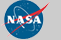Curator: David M. Giles NASA Official: Brent N. Holben Generated: 11/12/2019